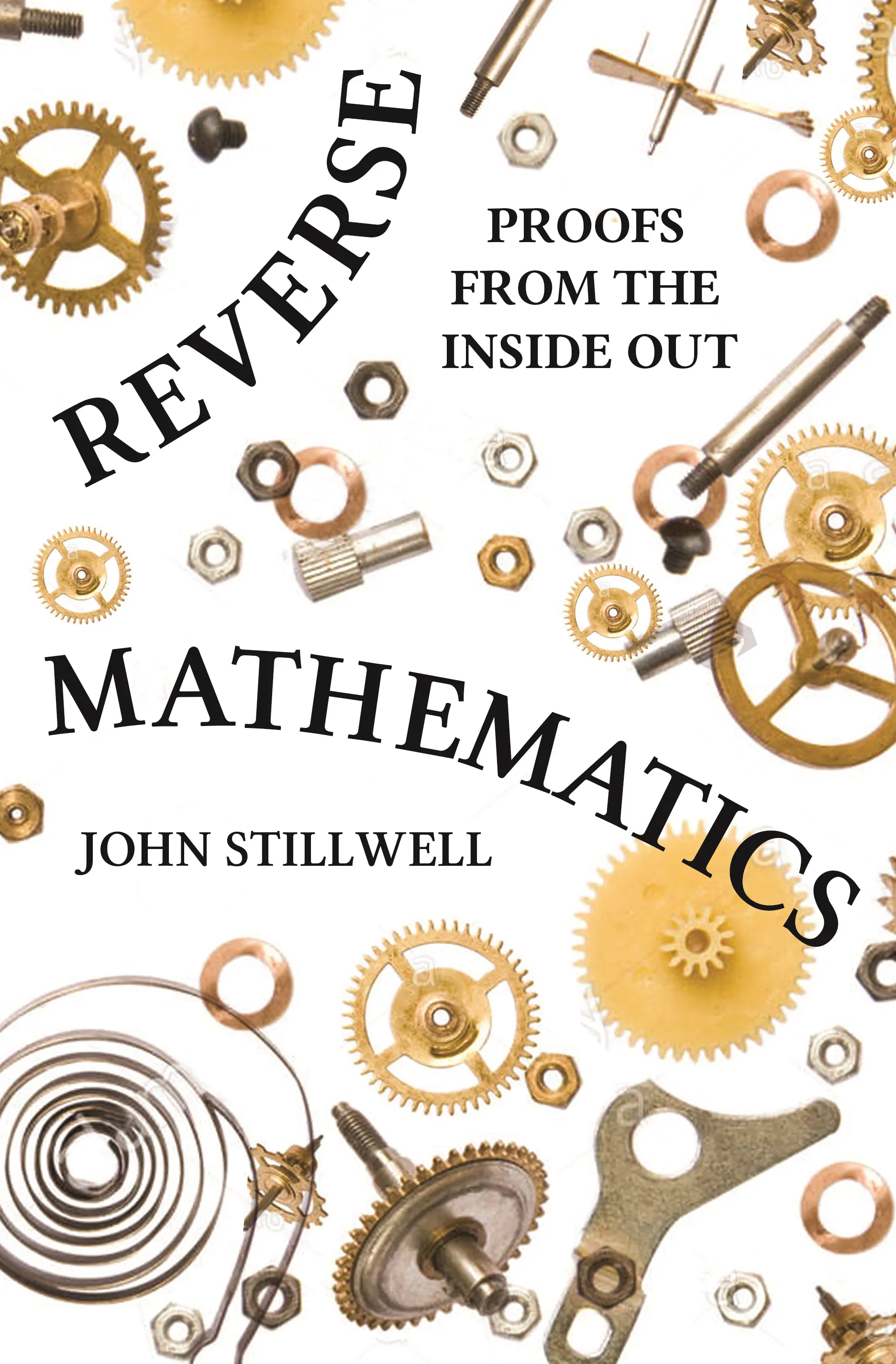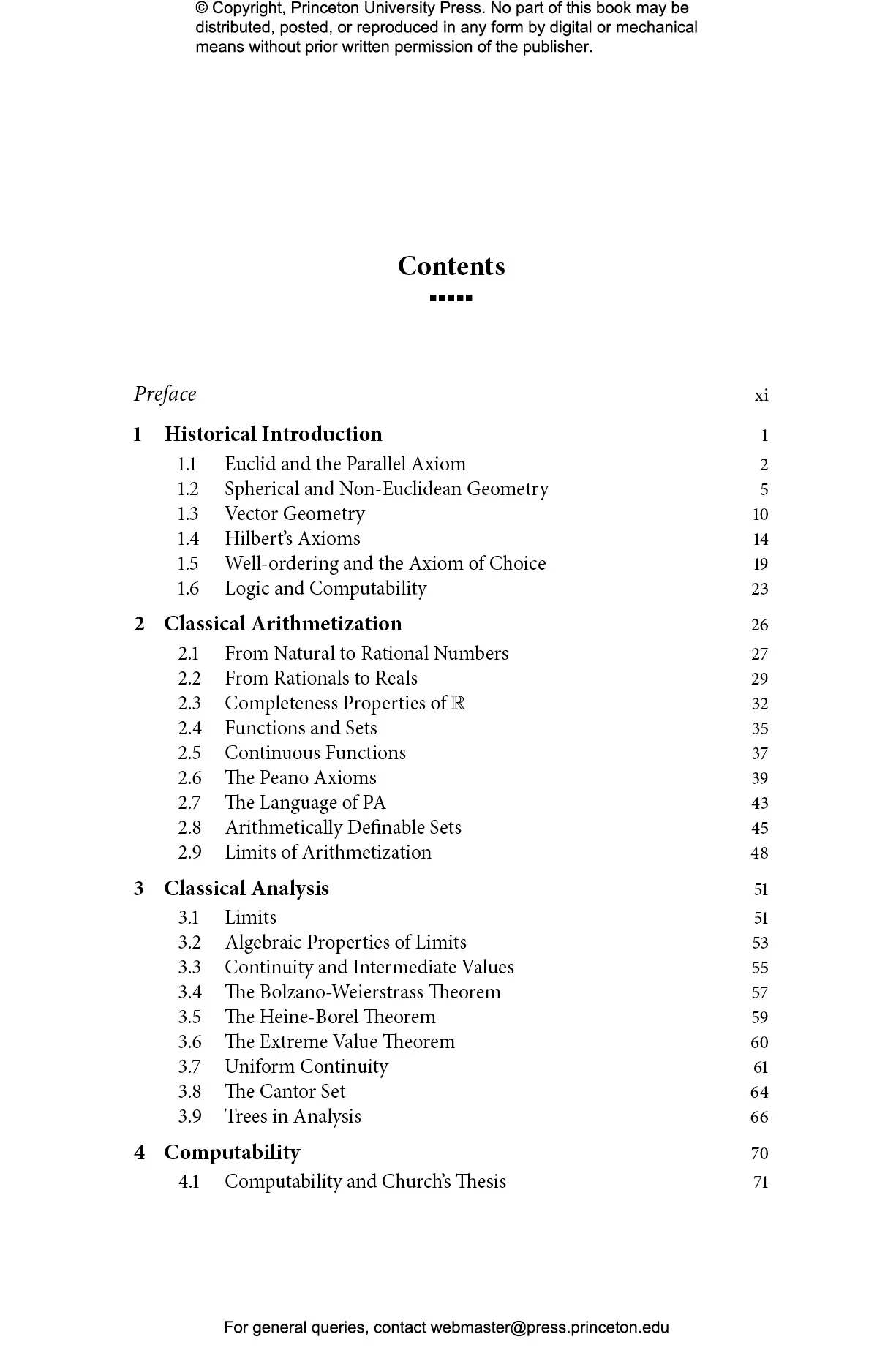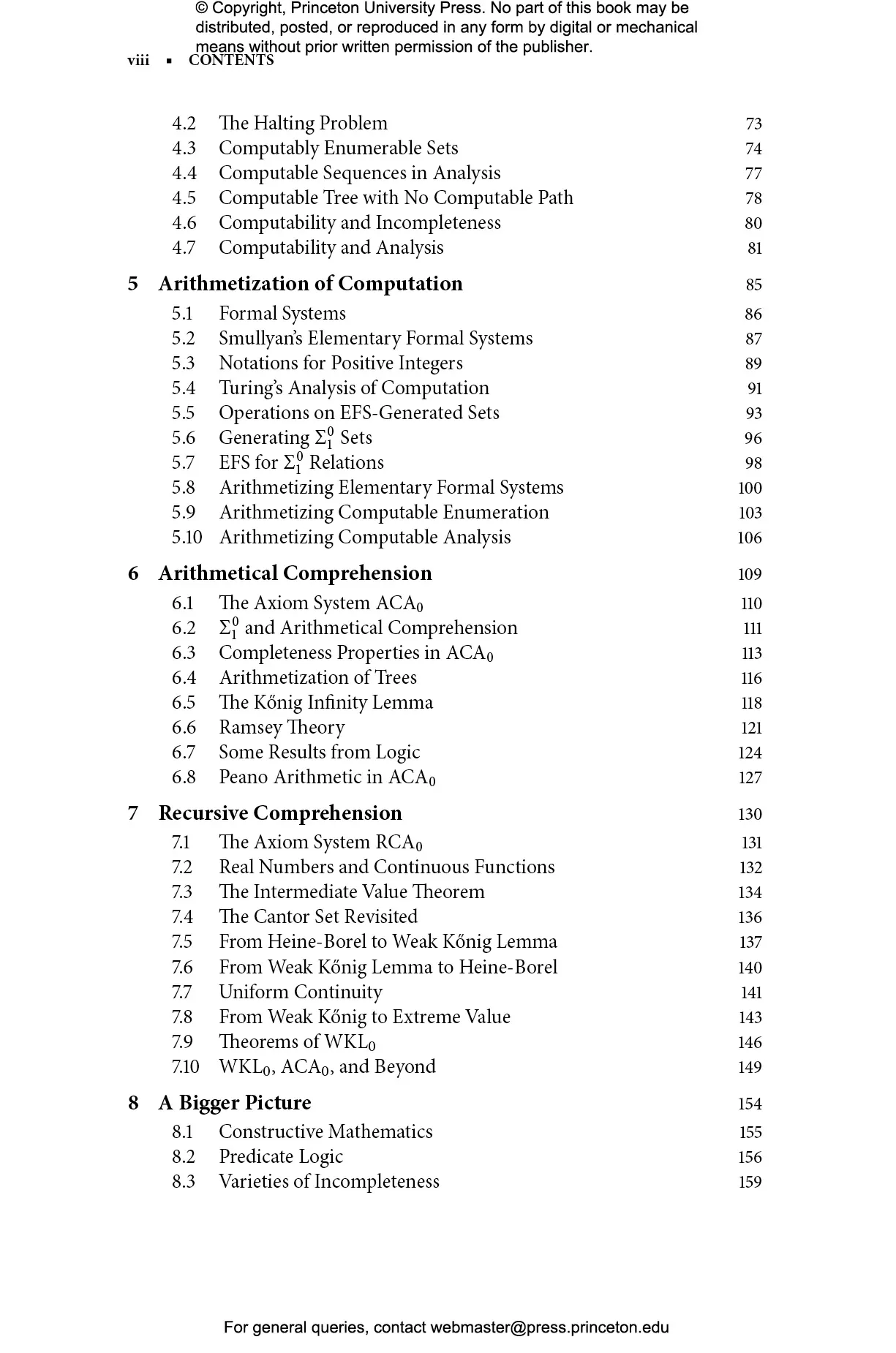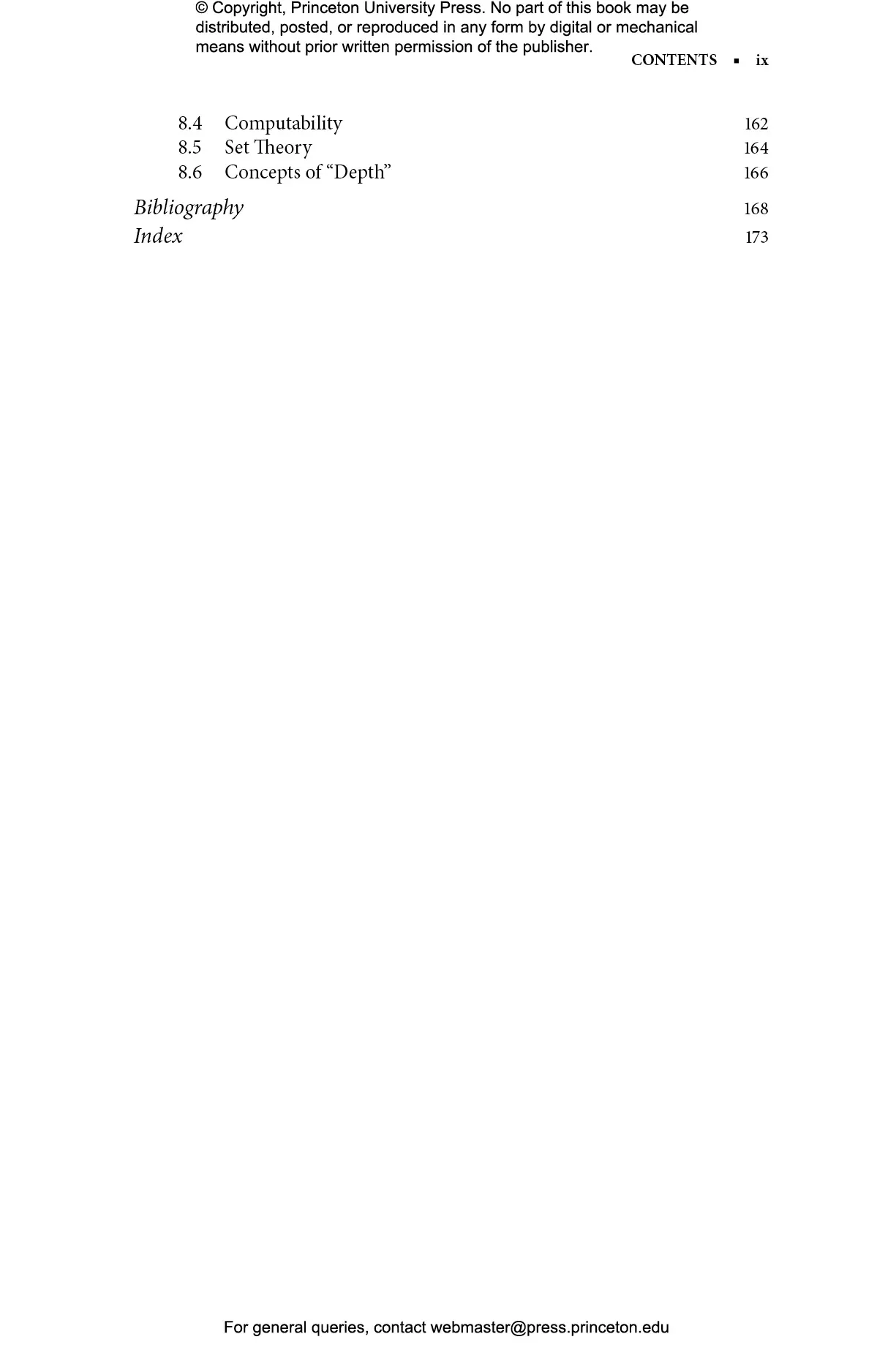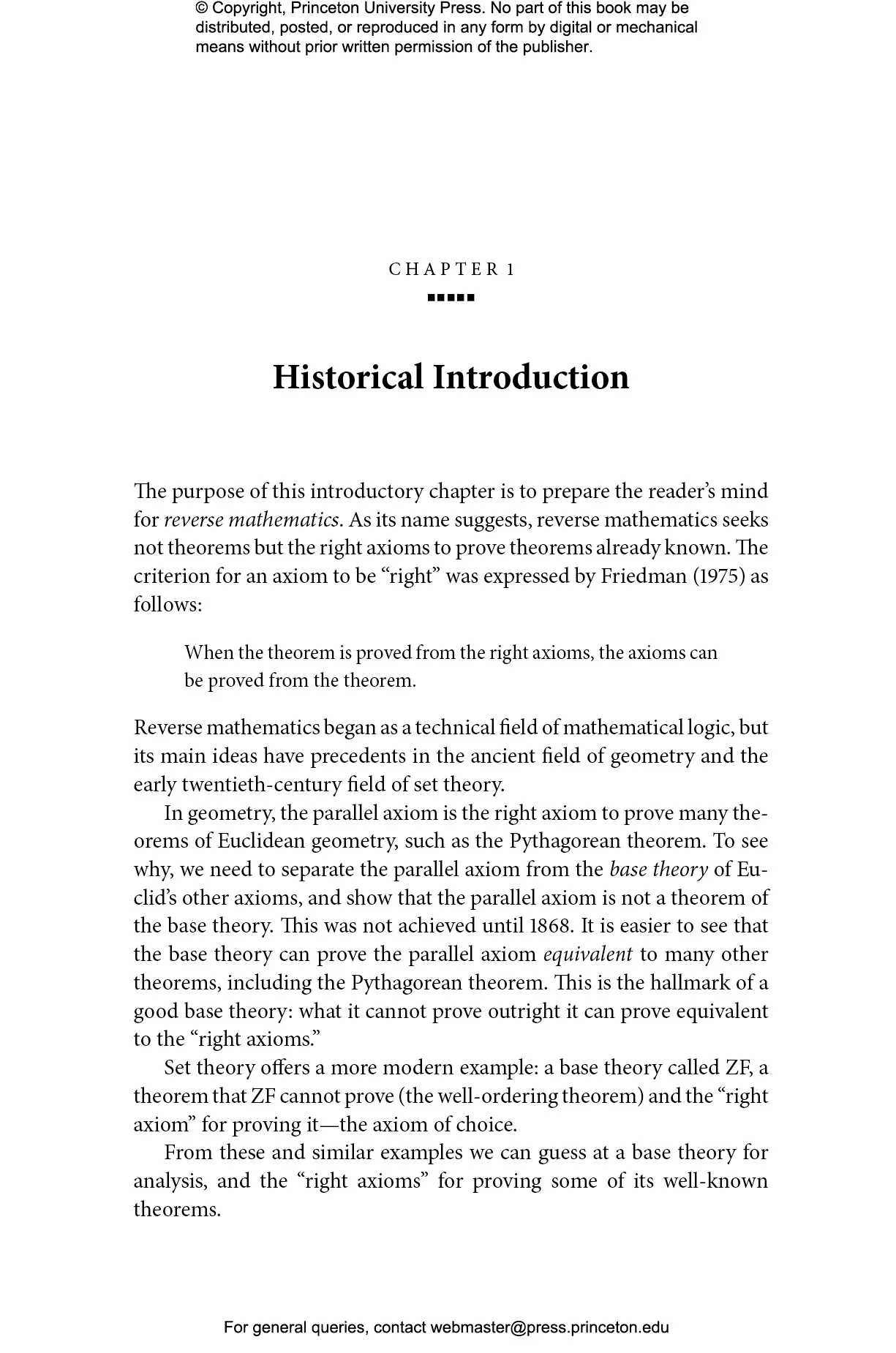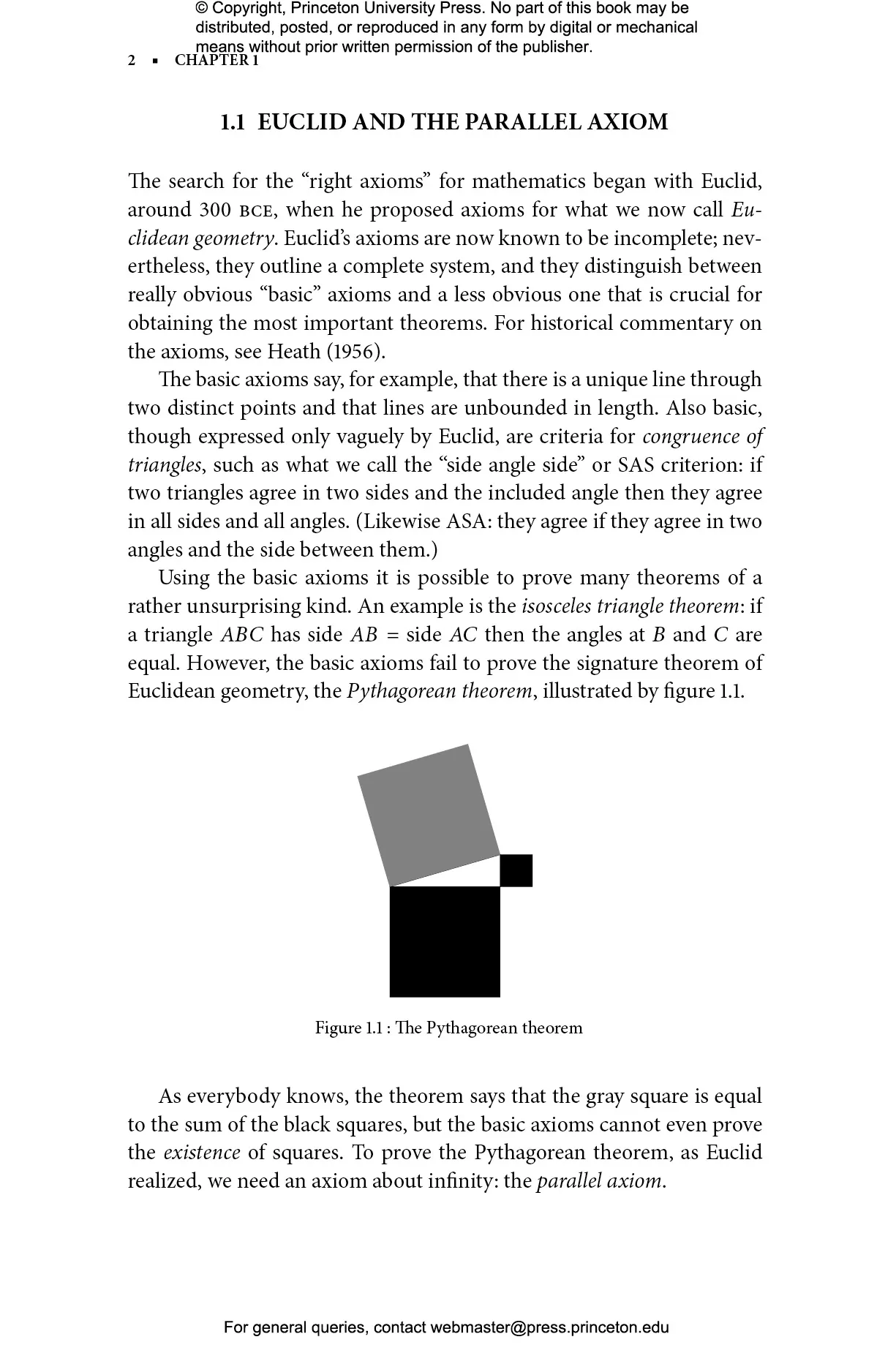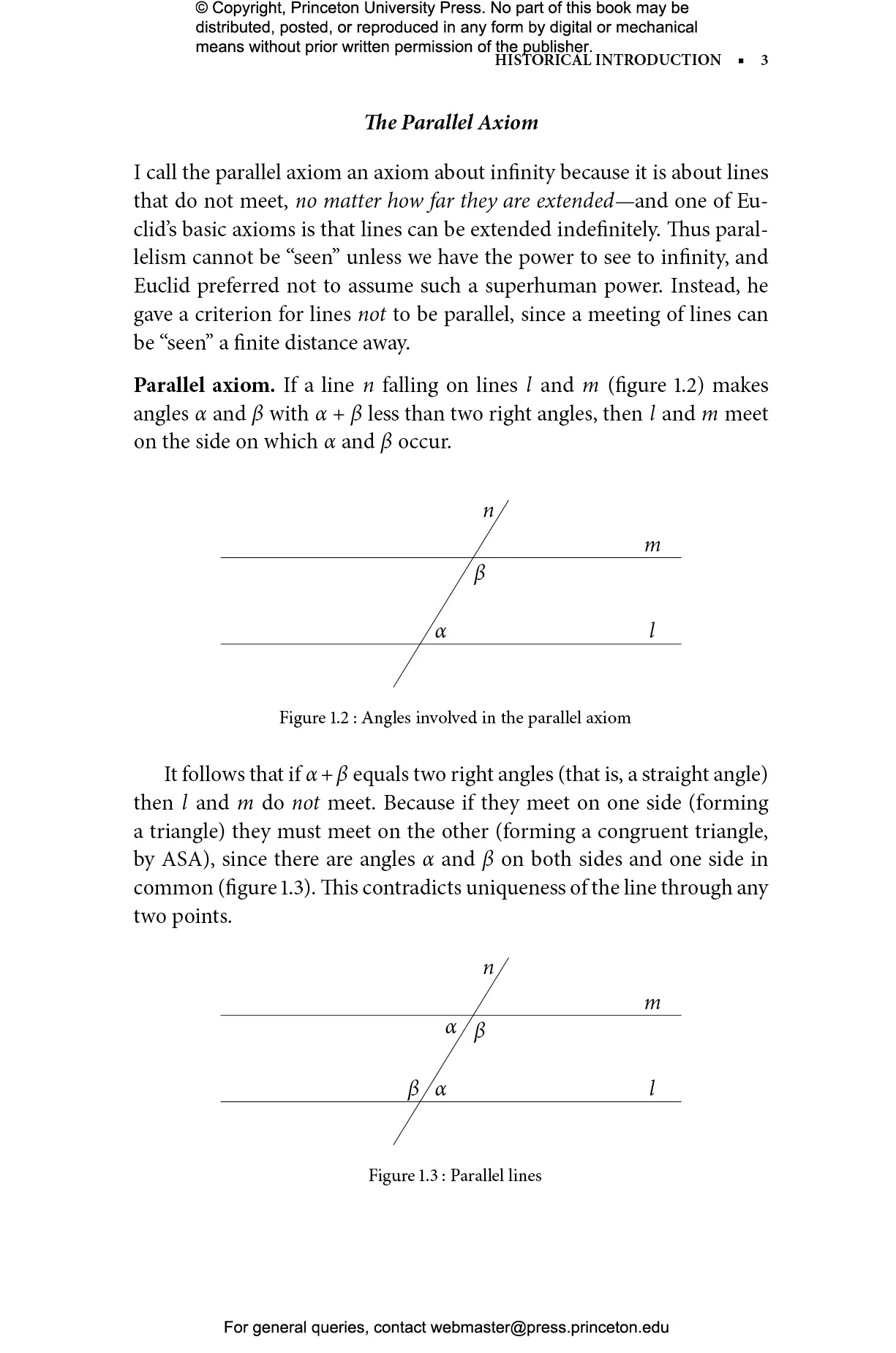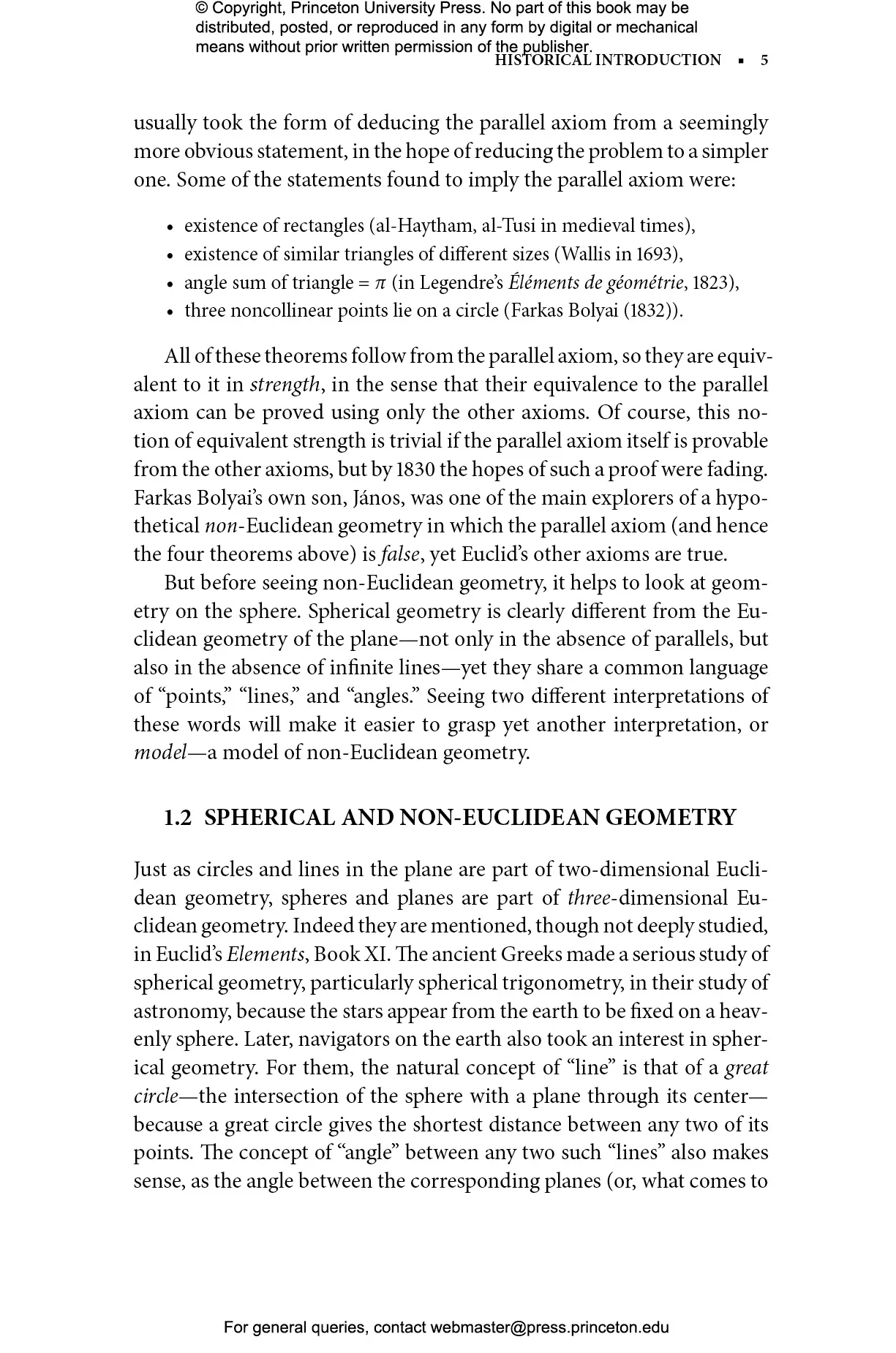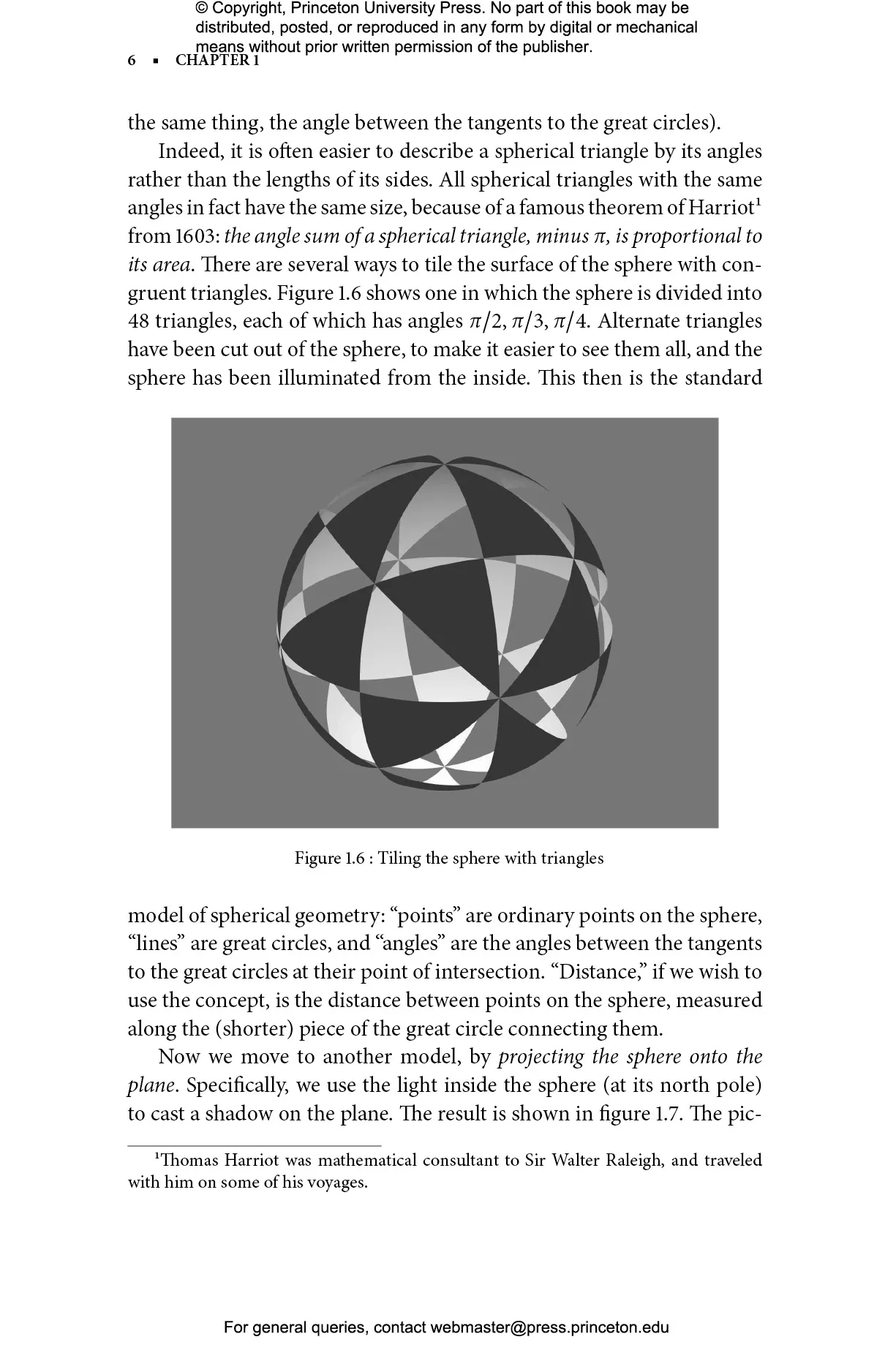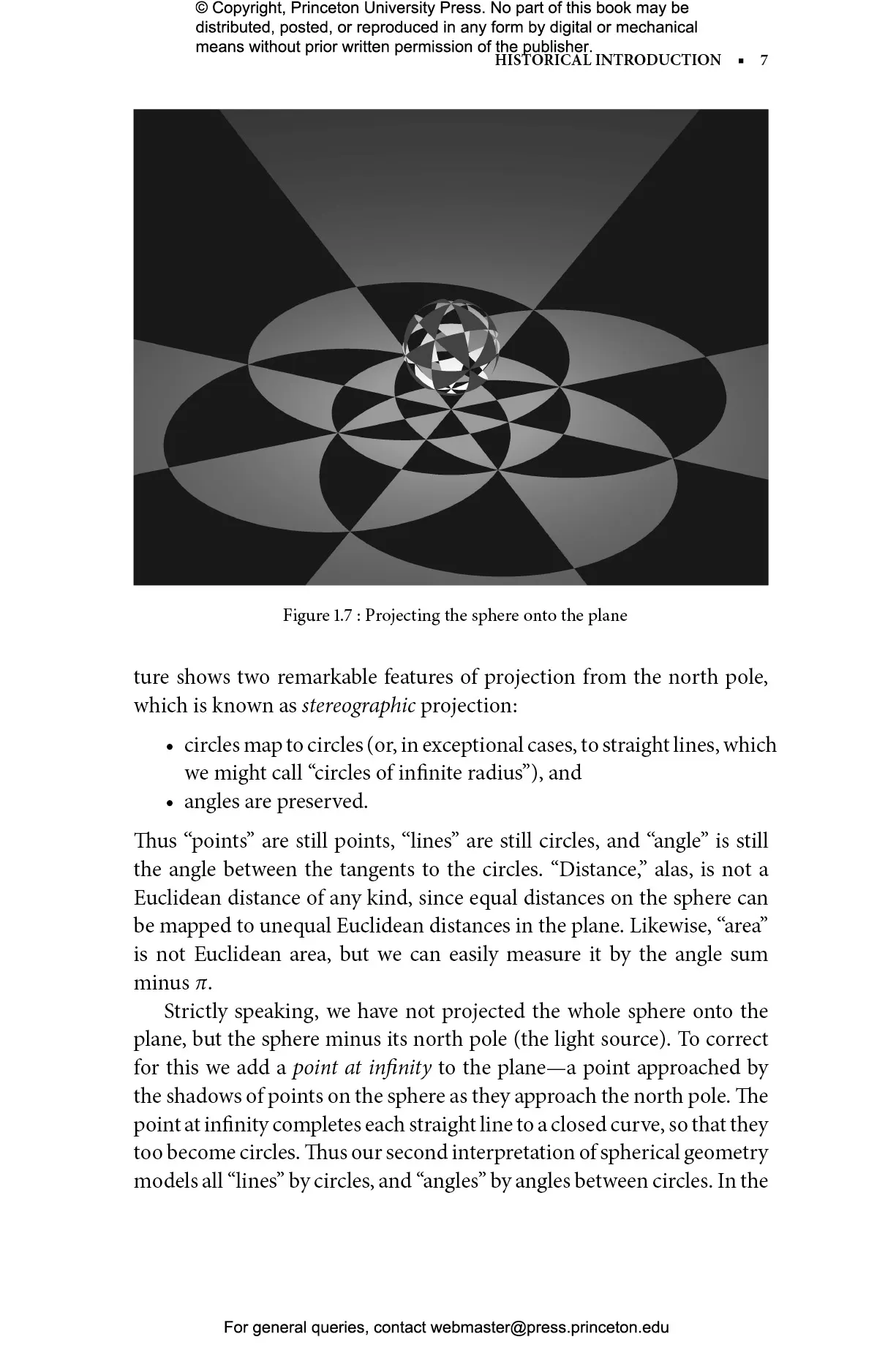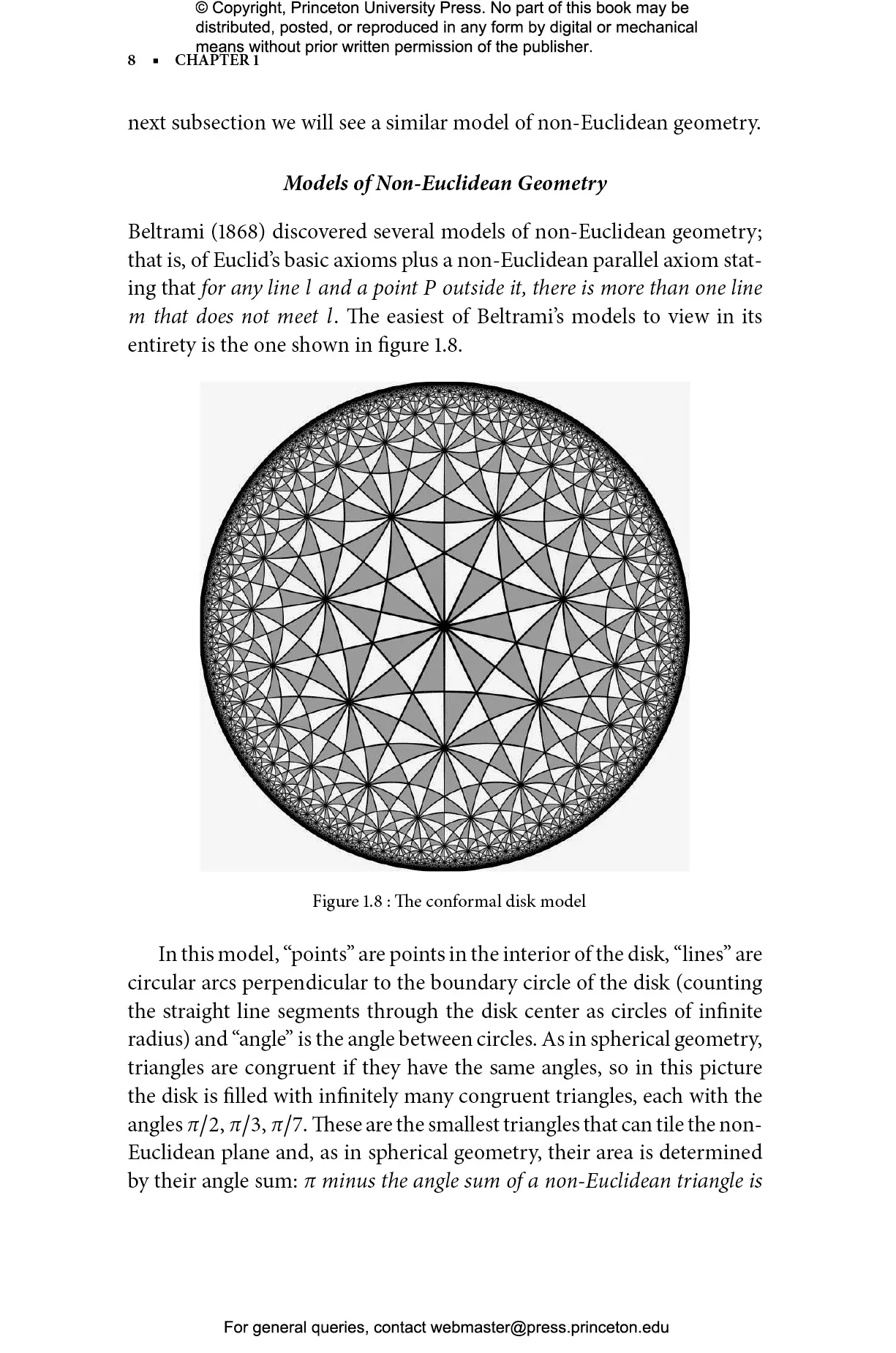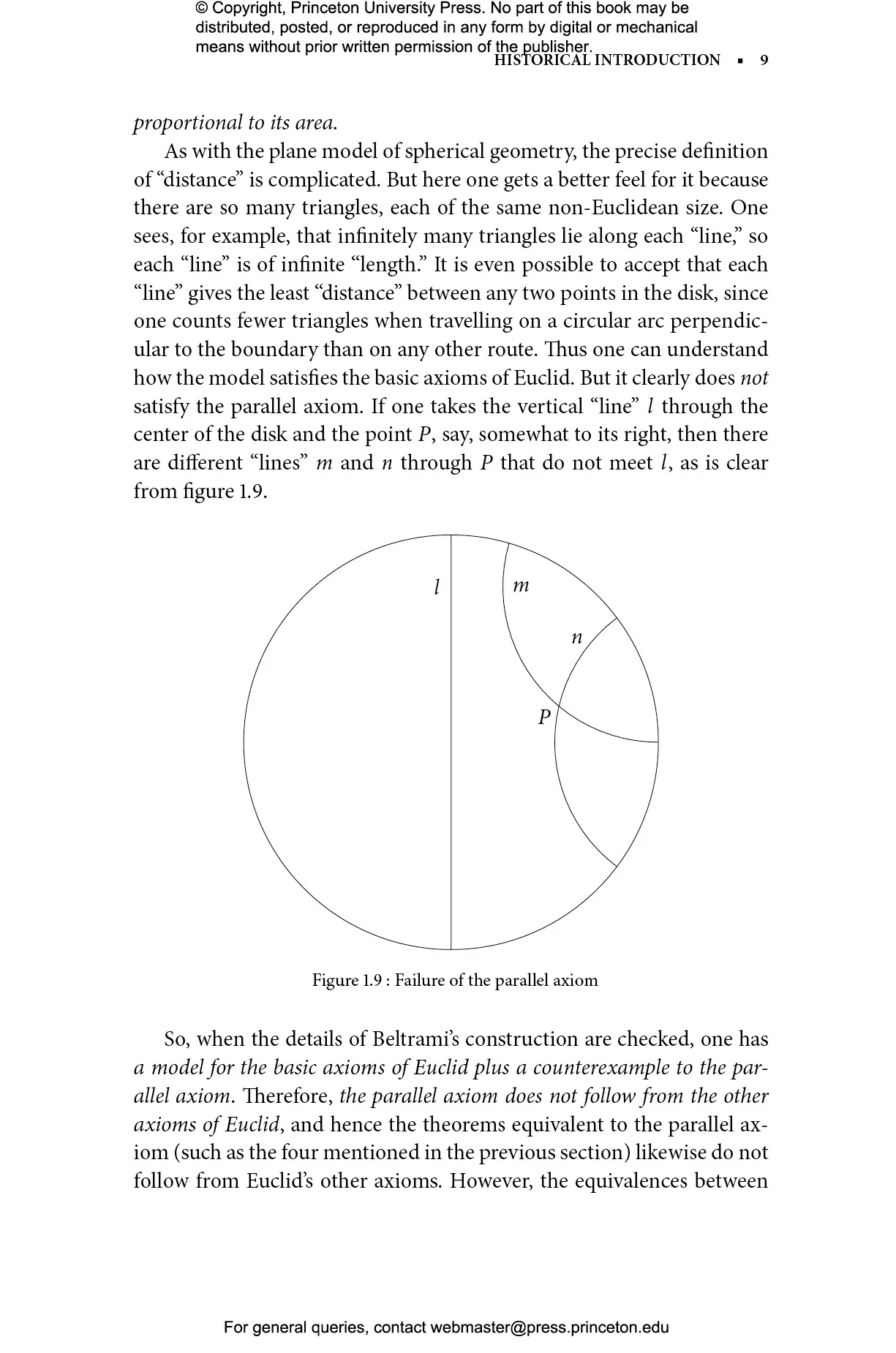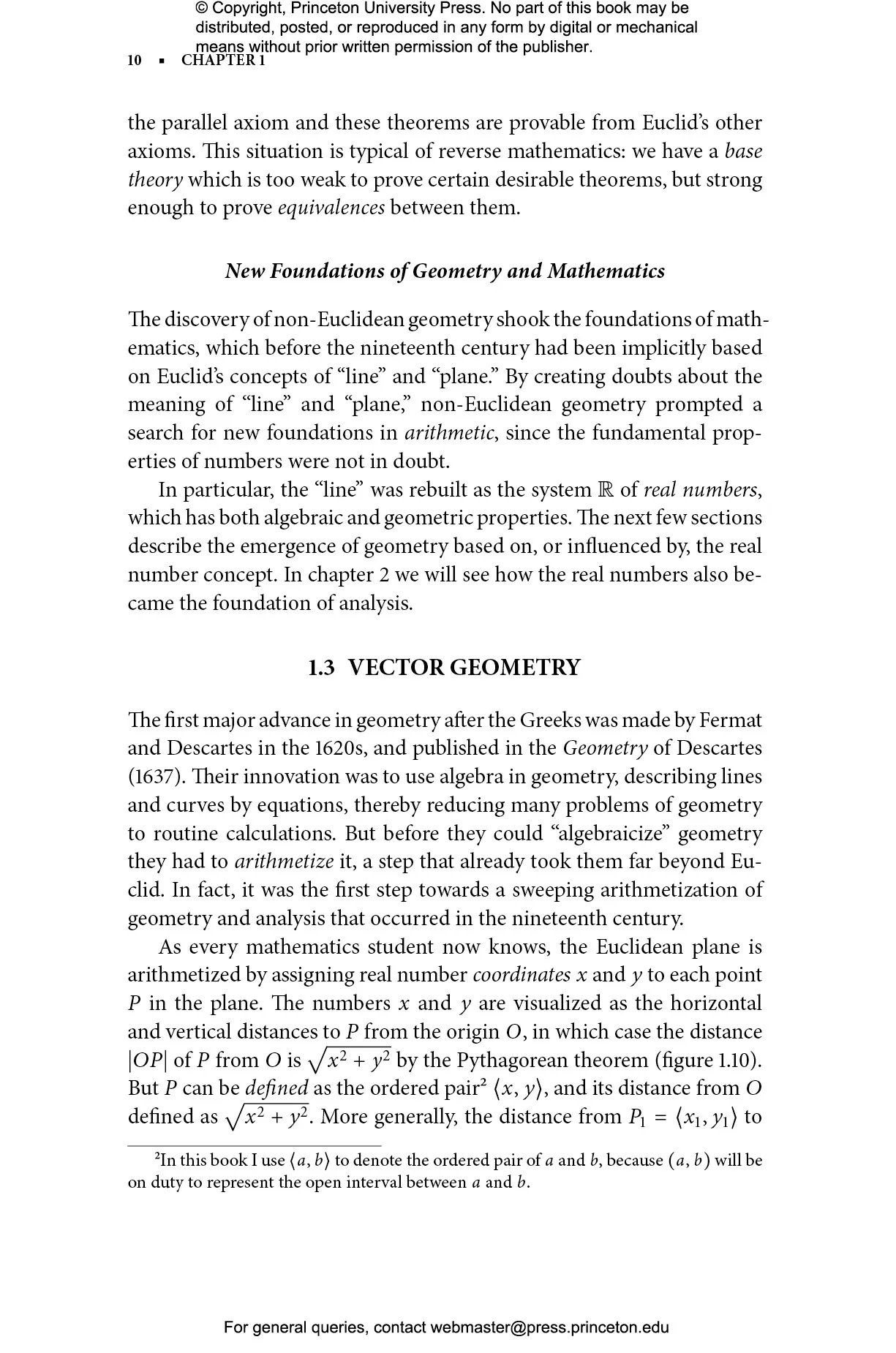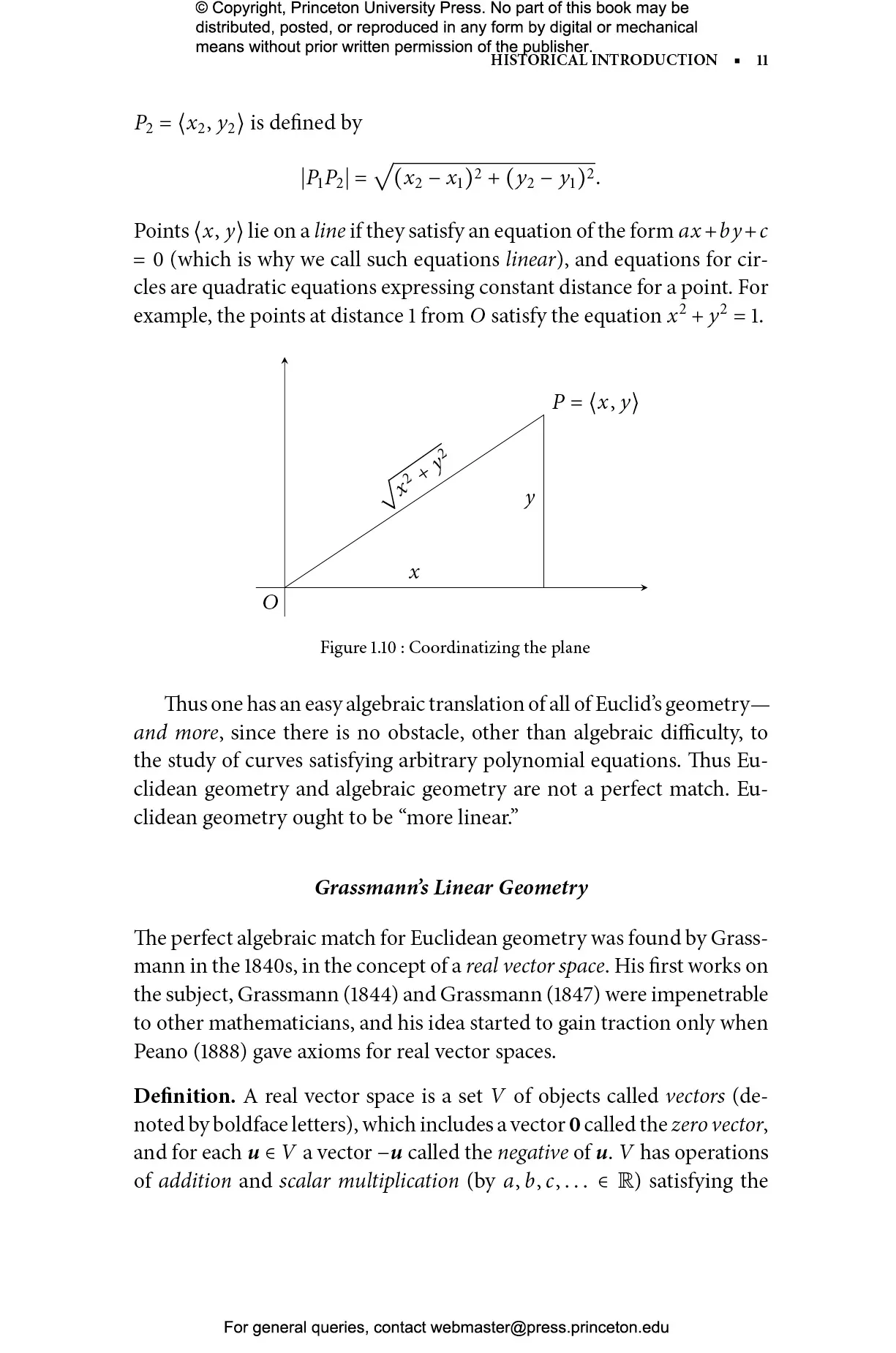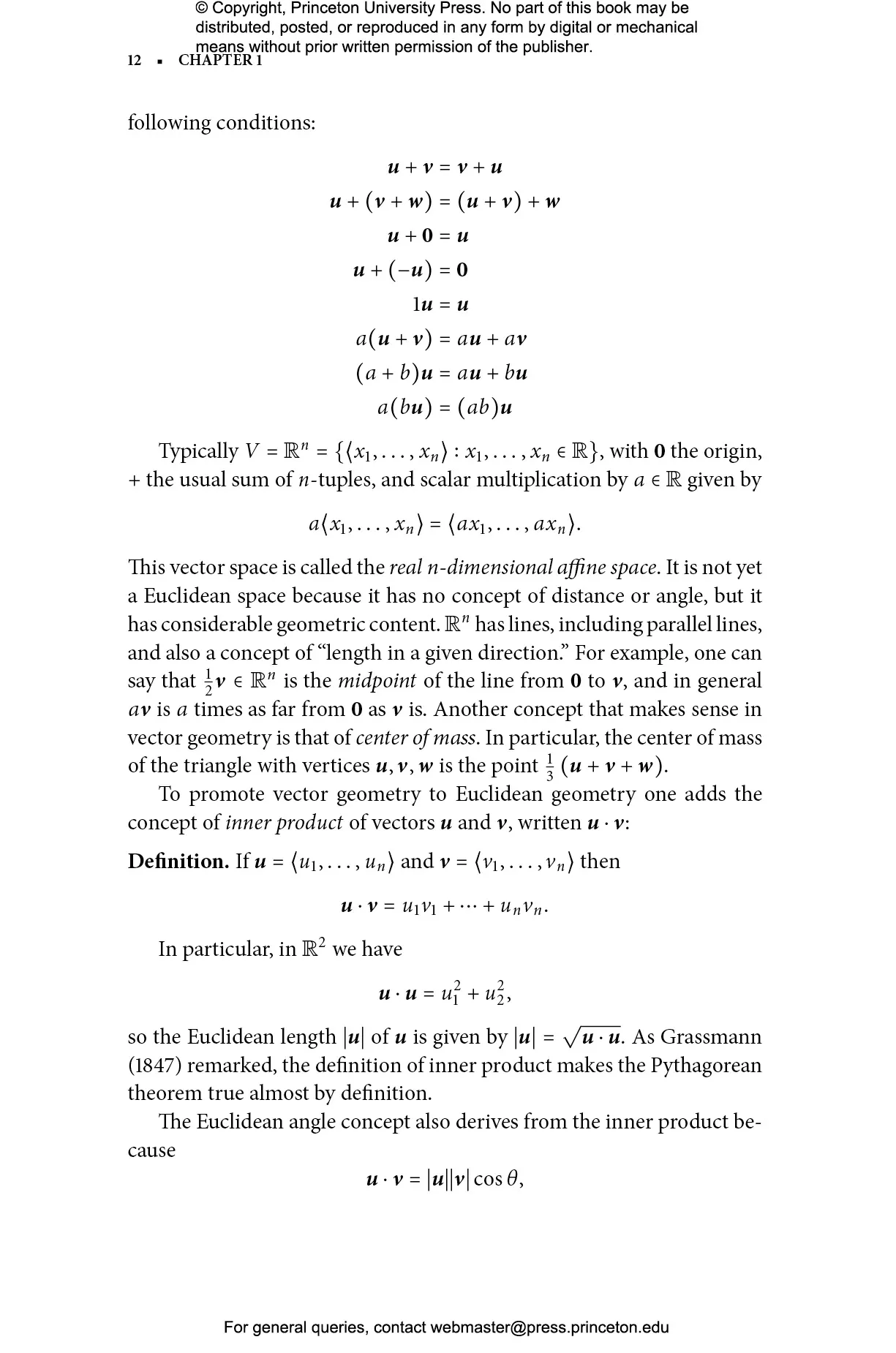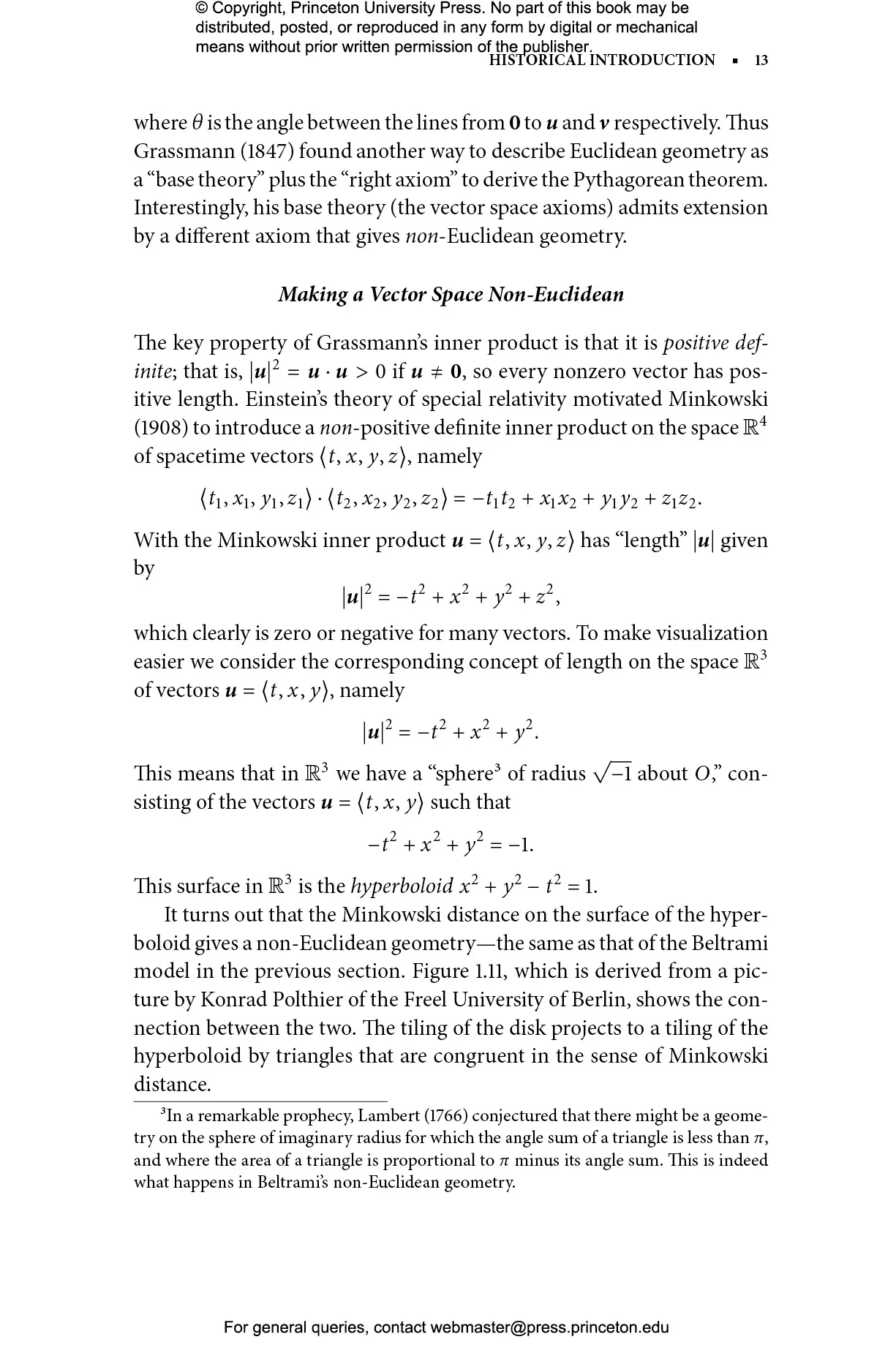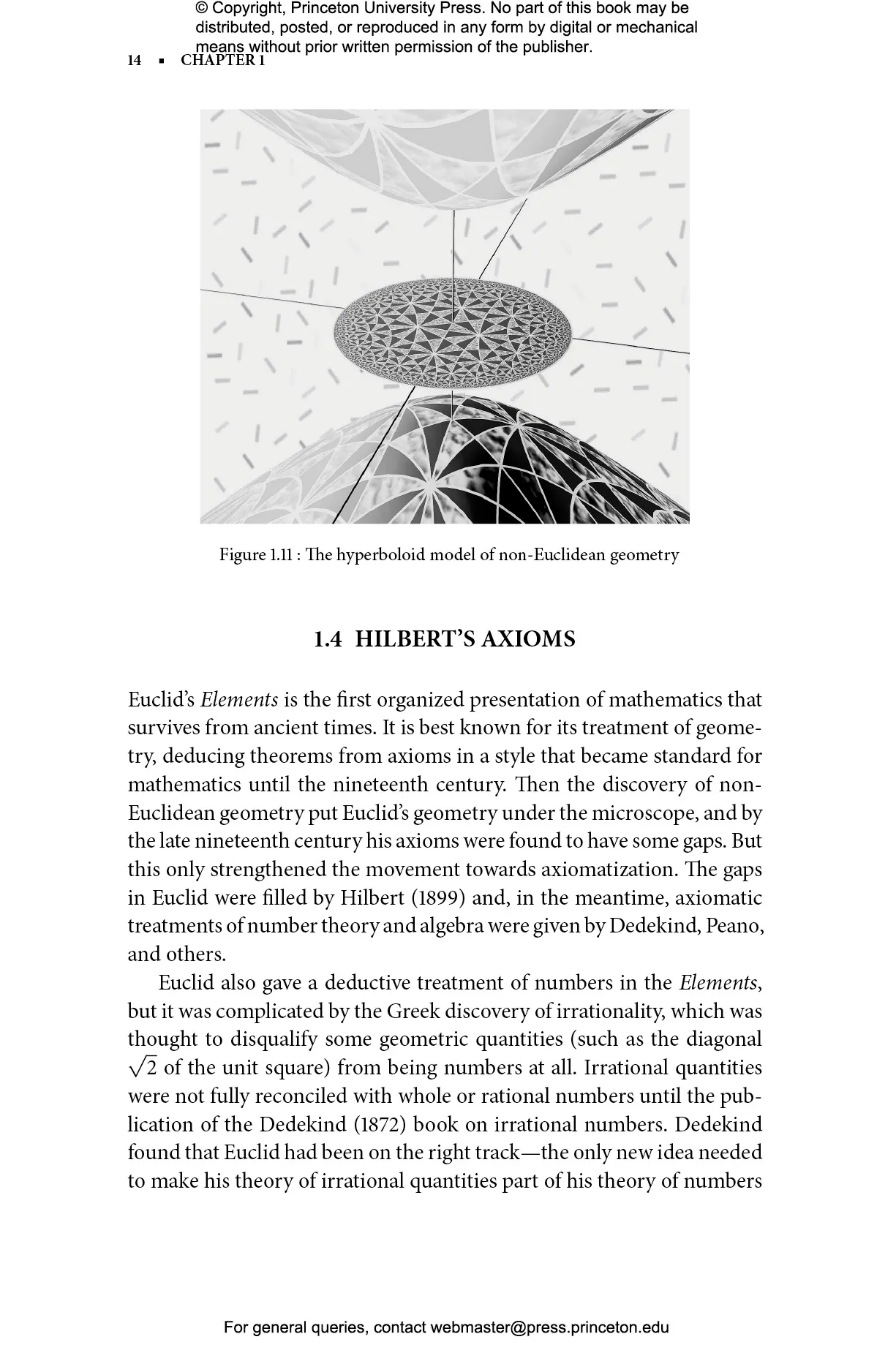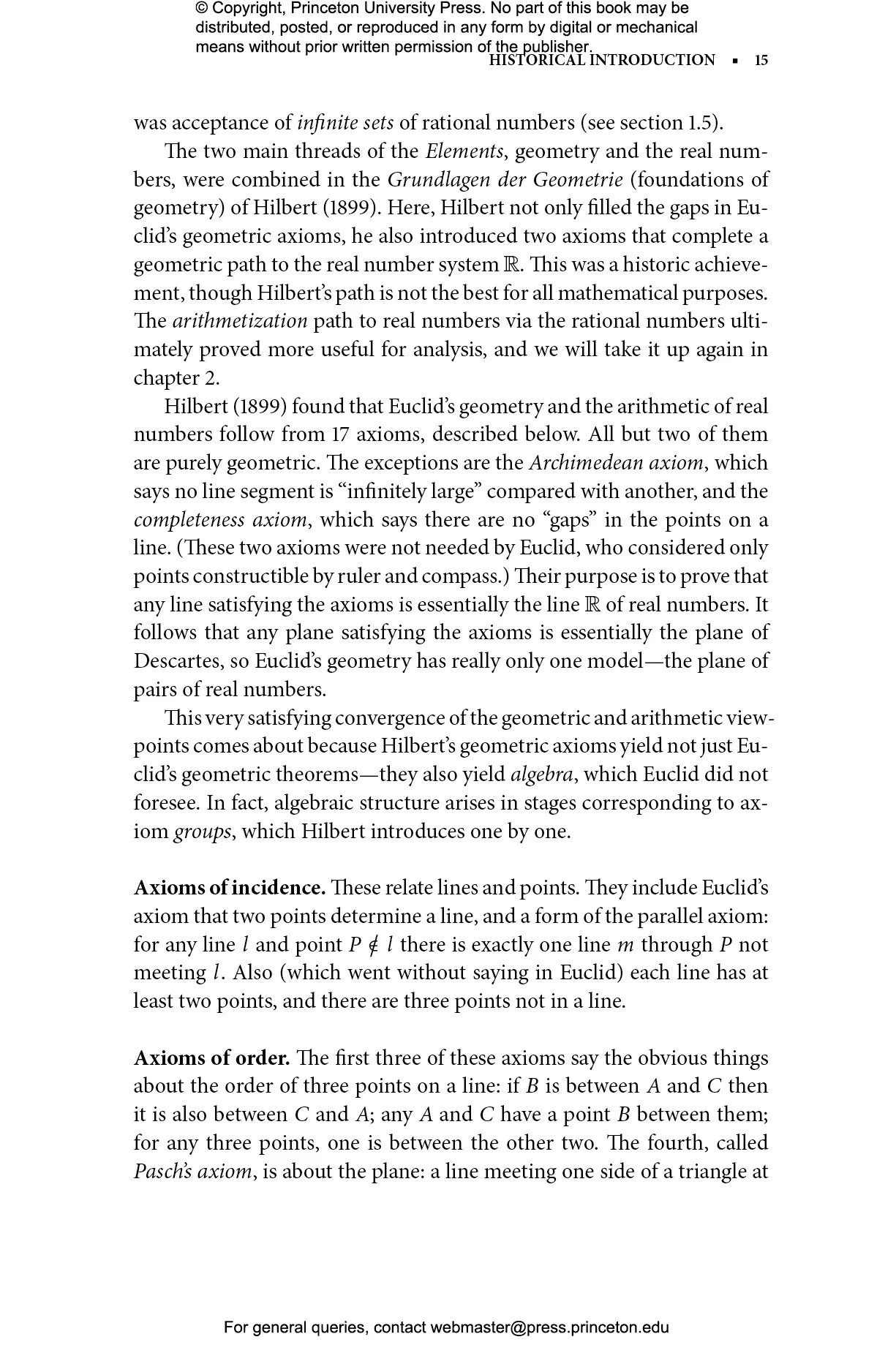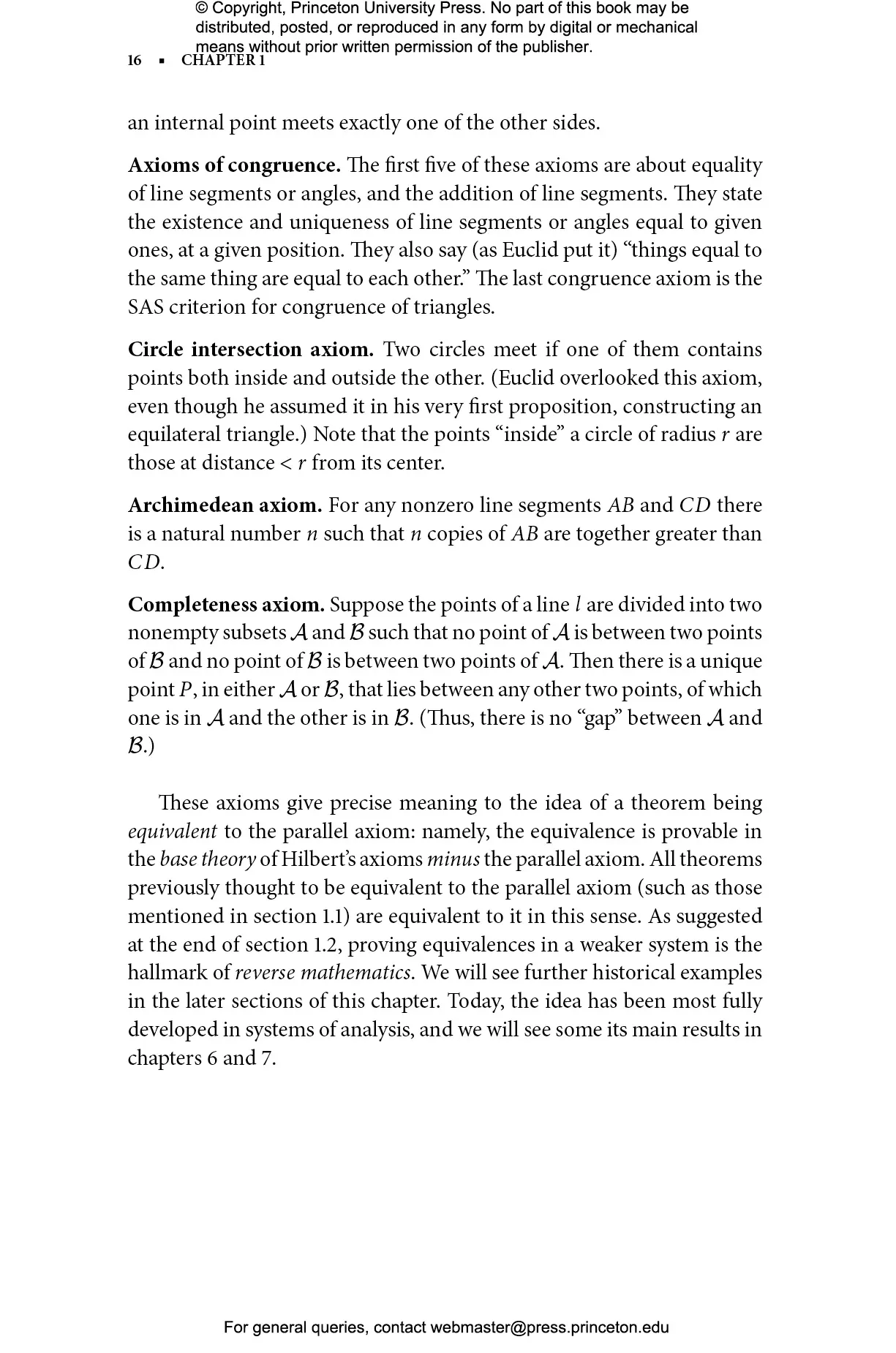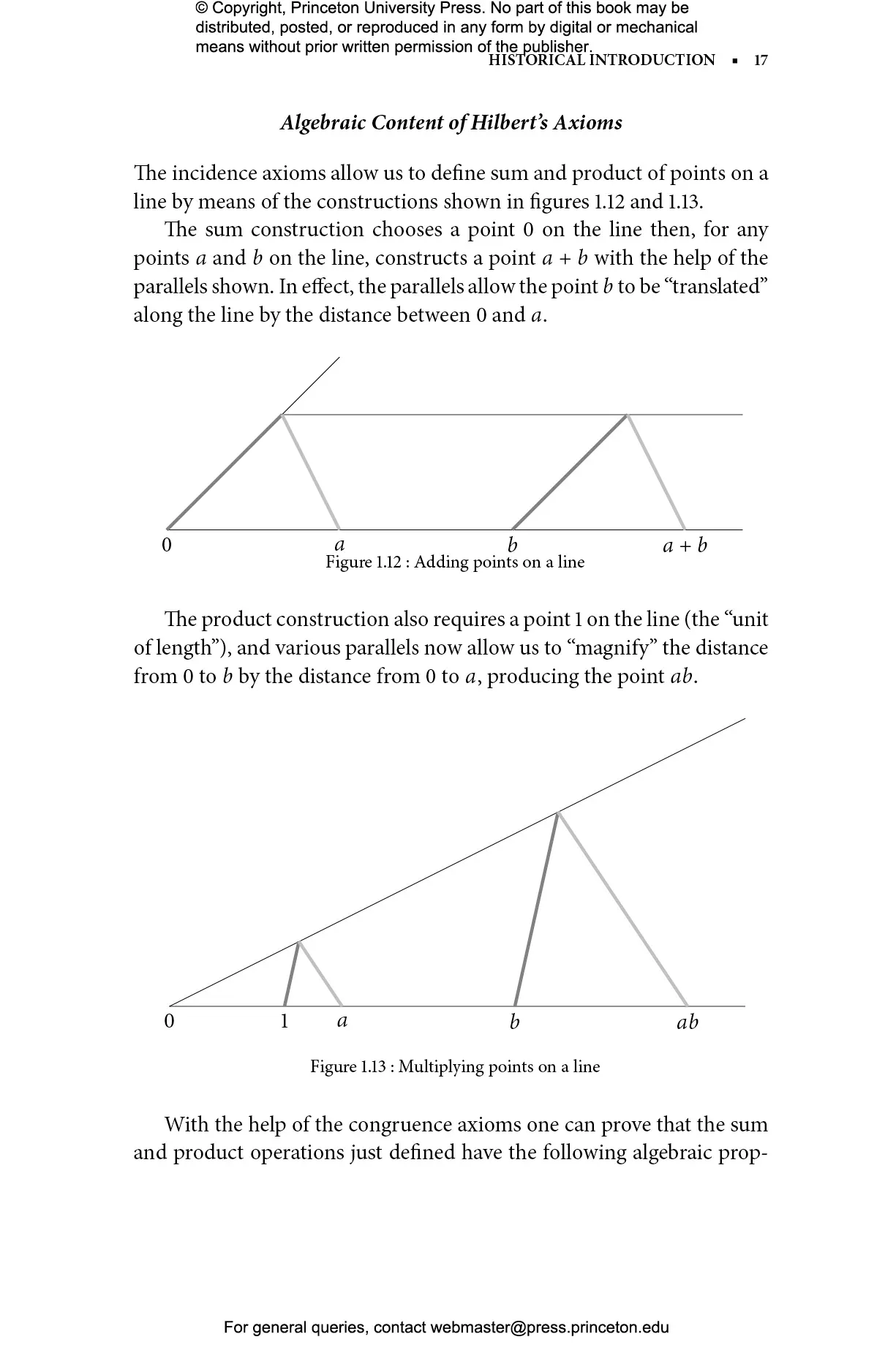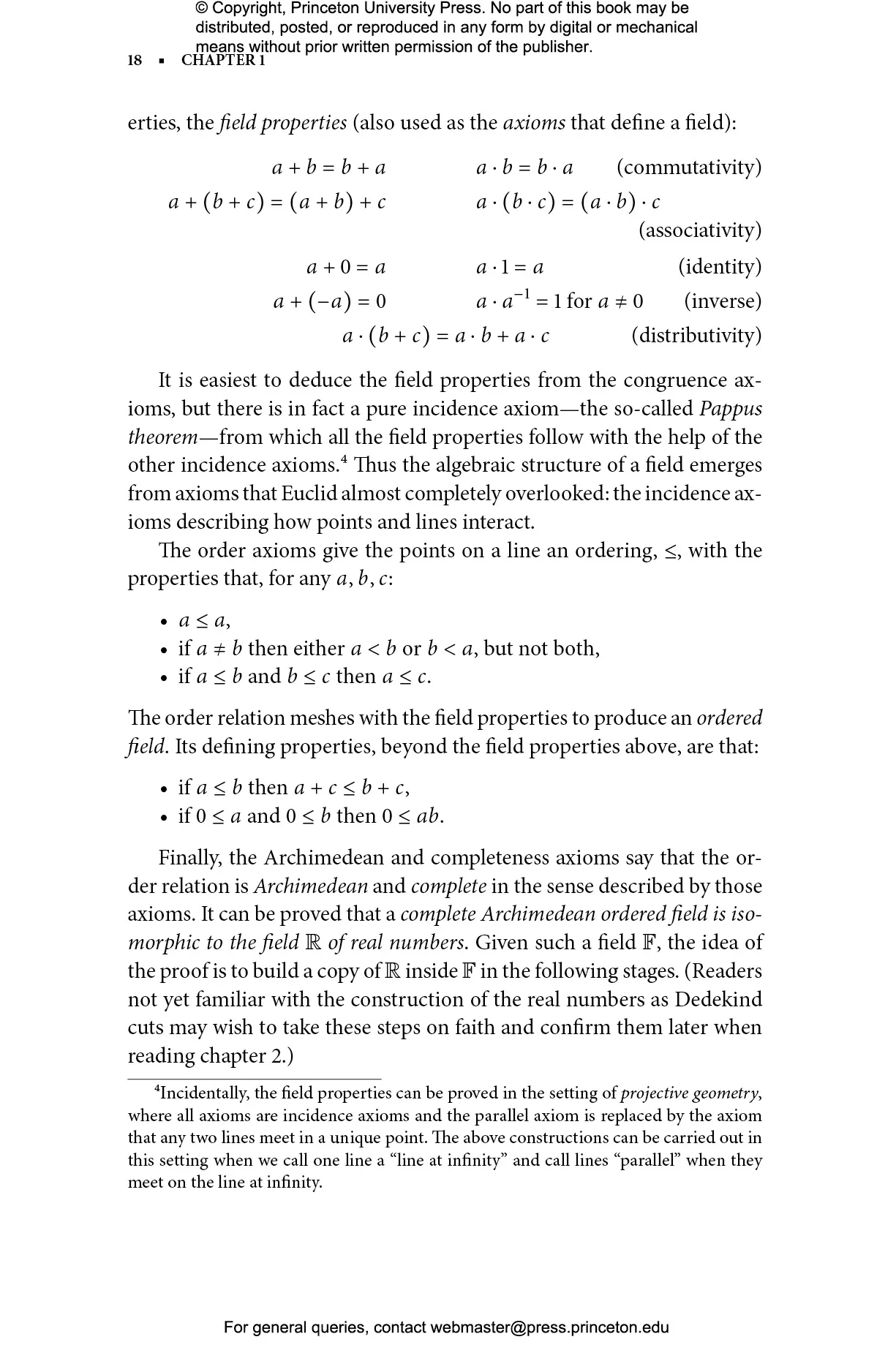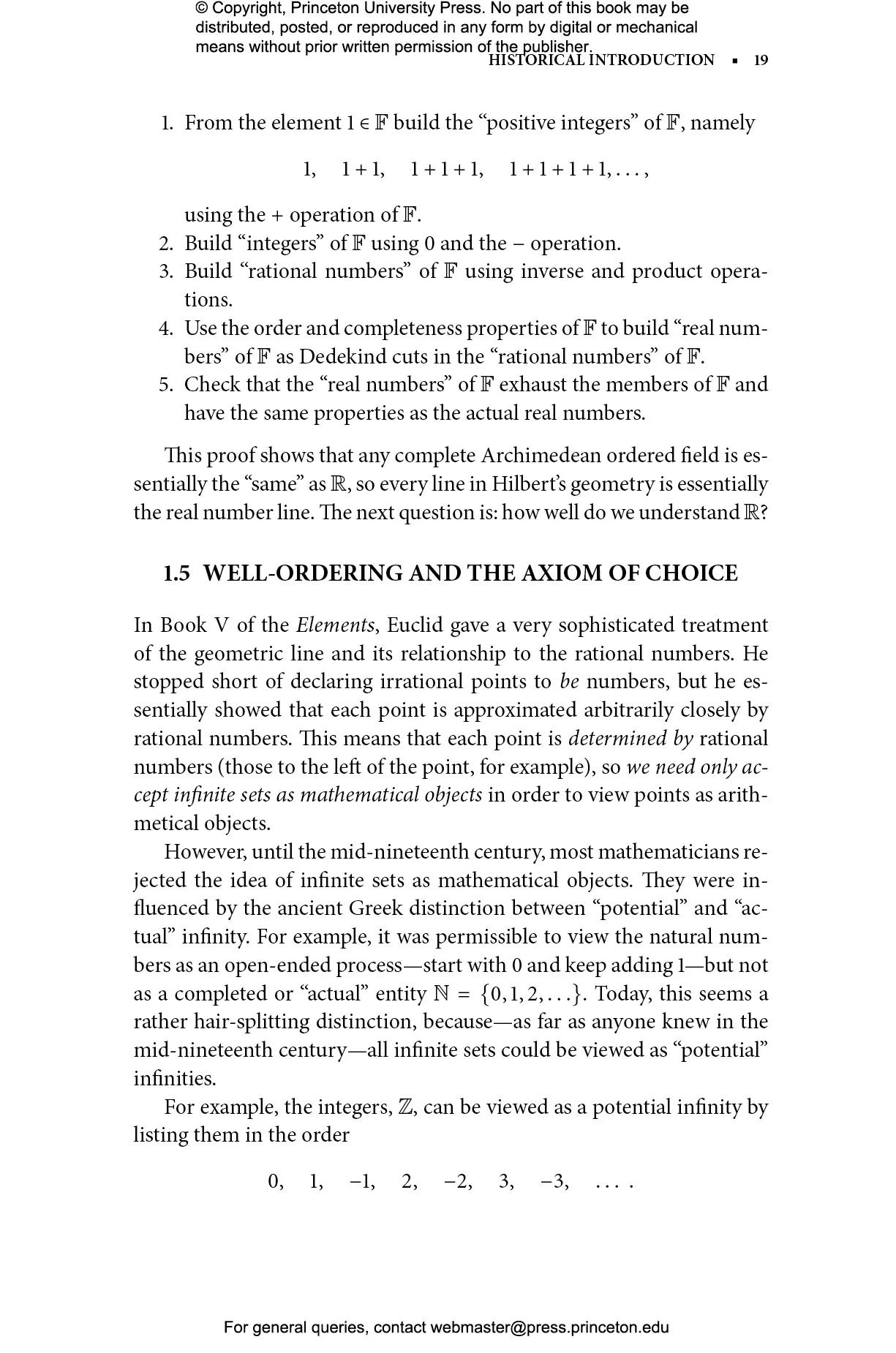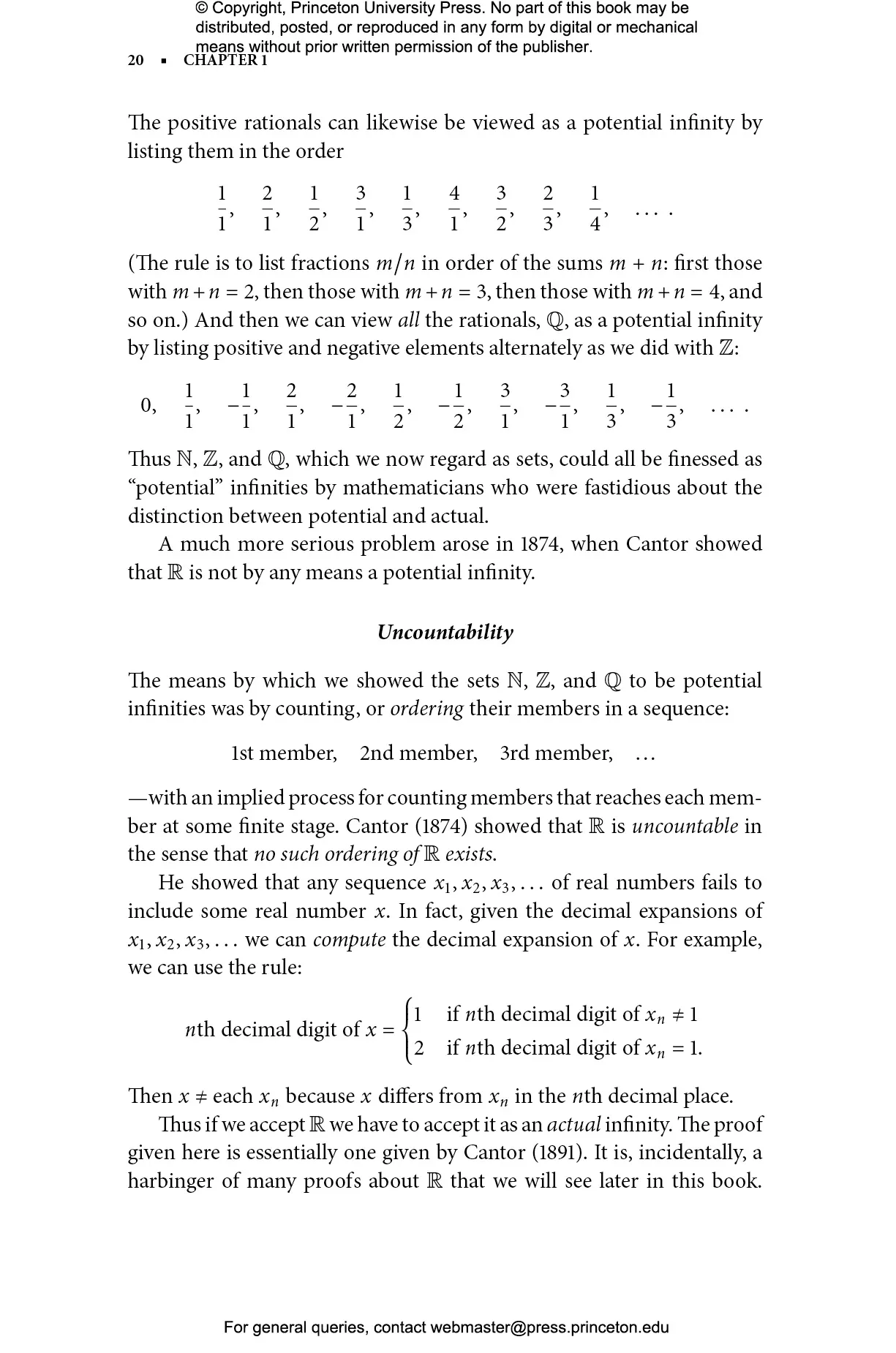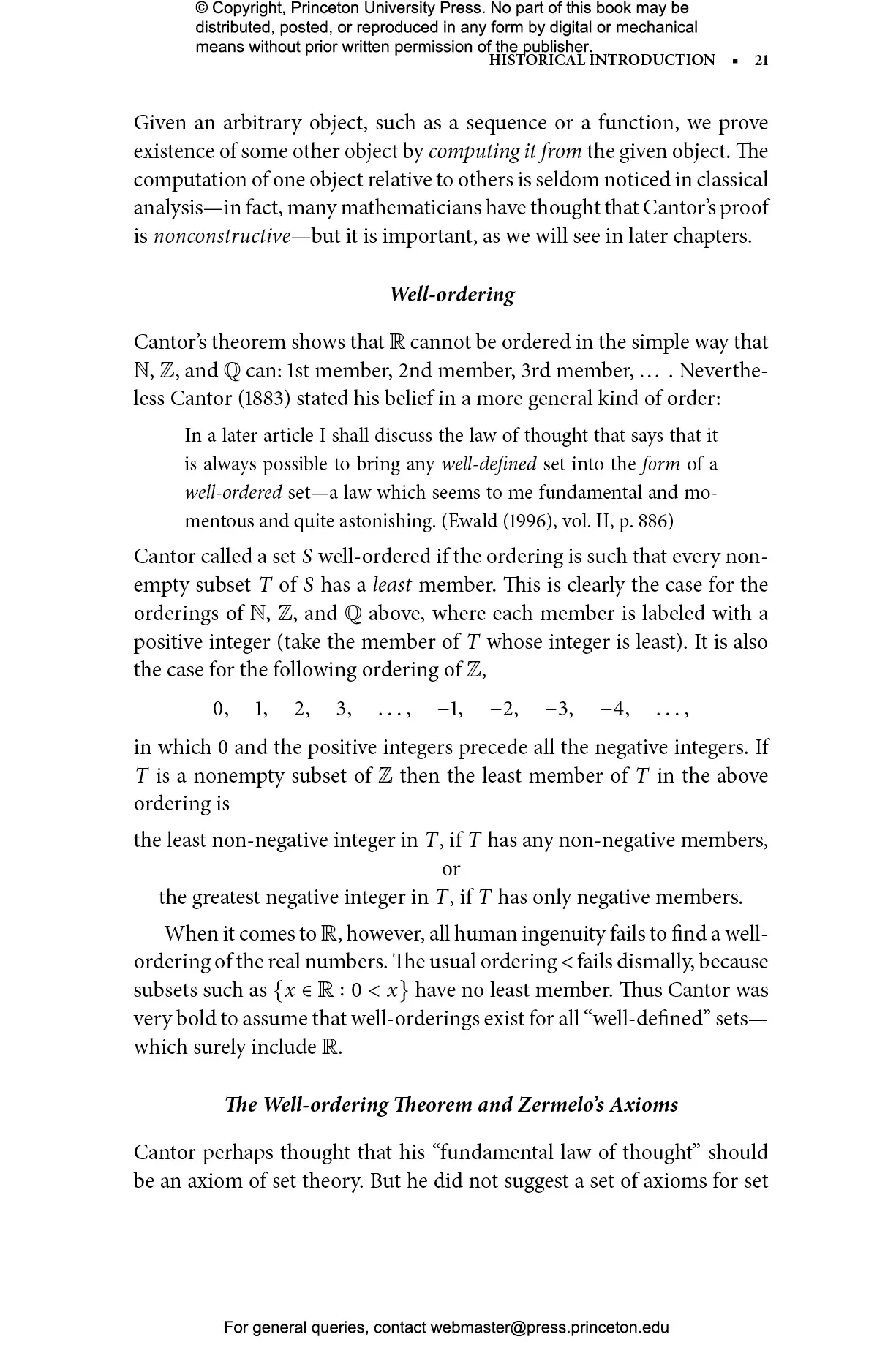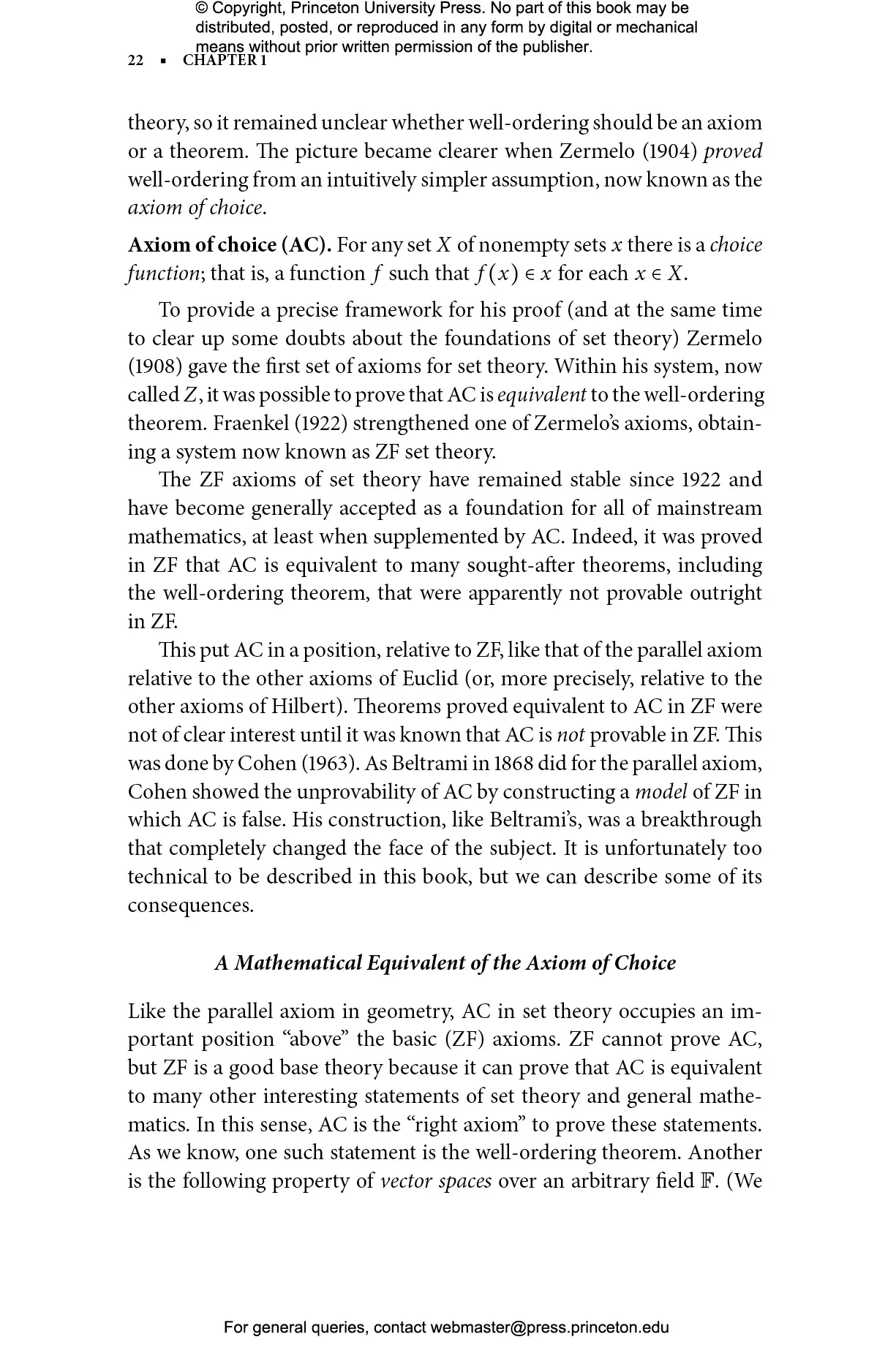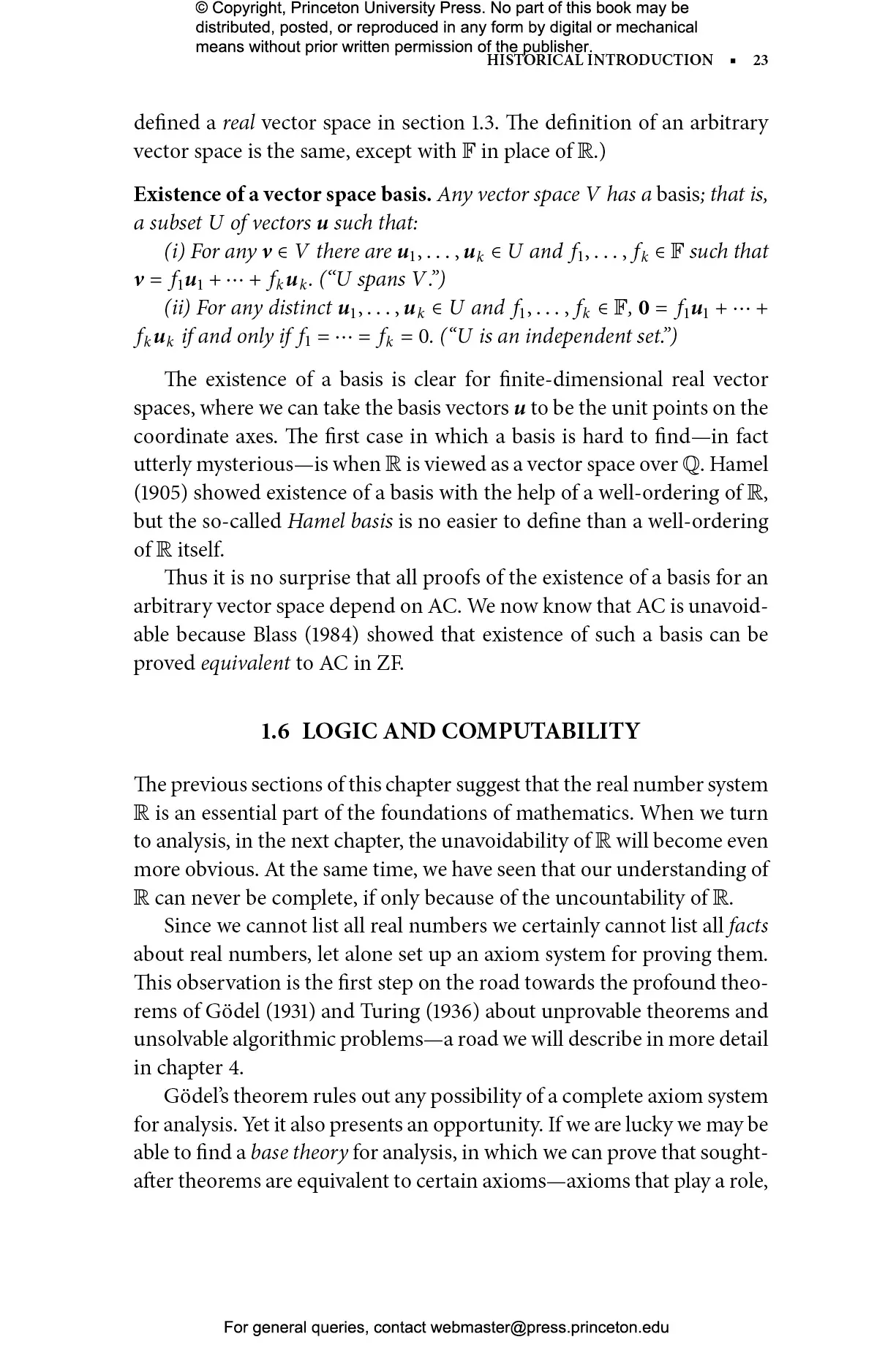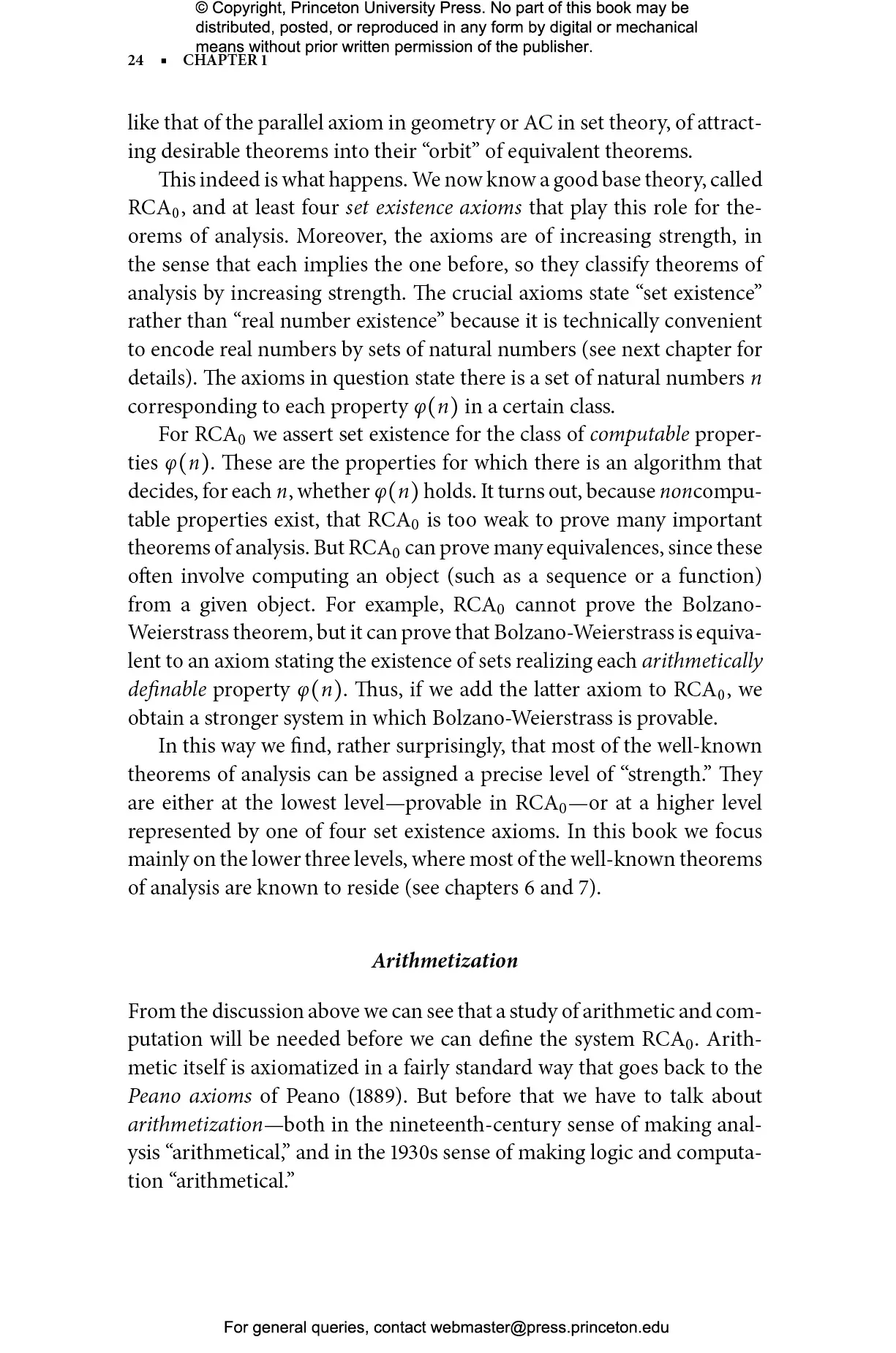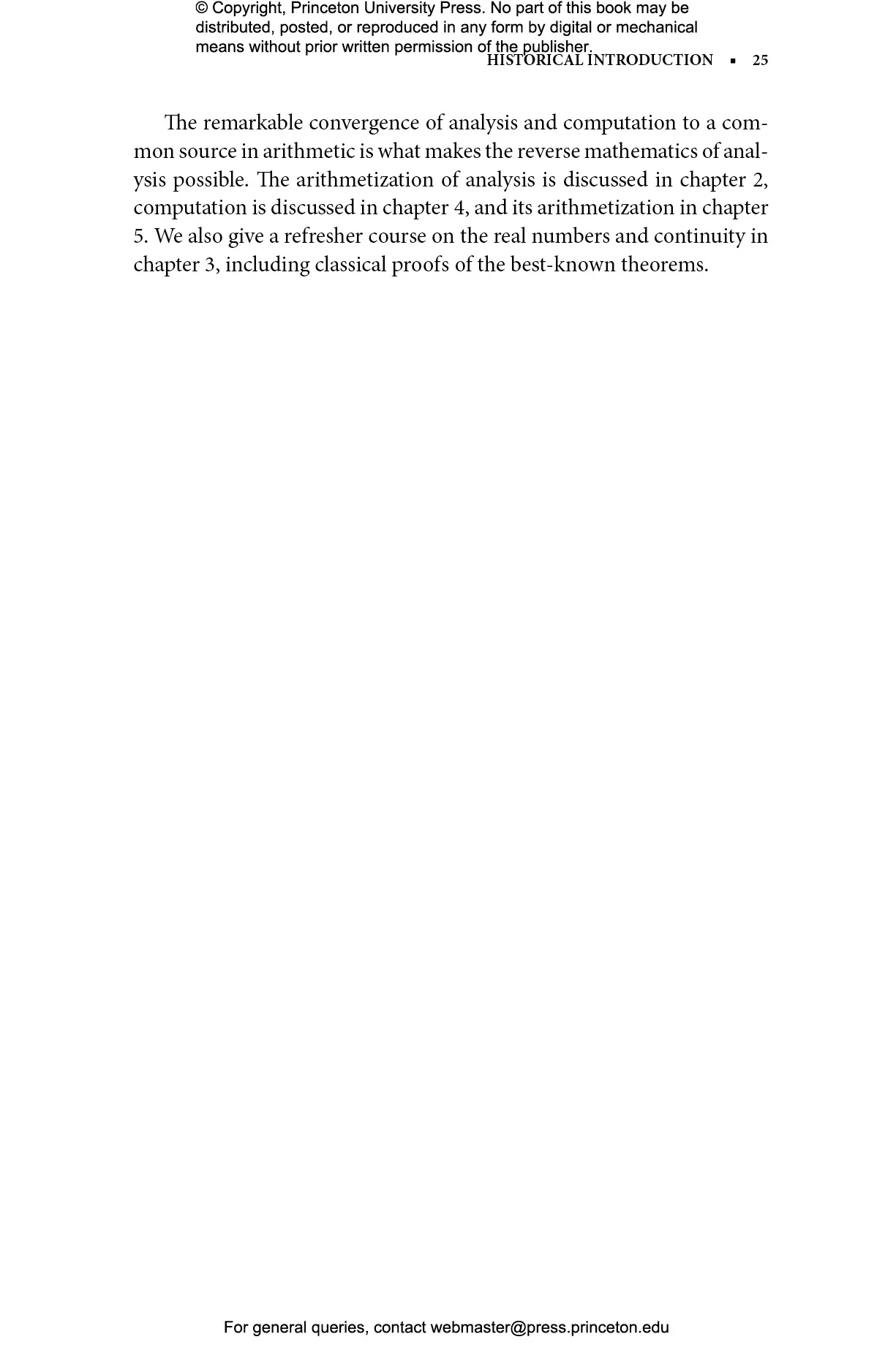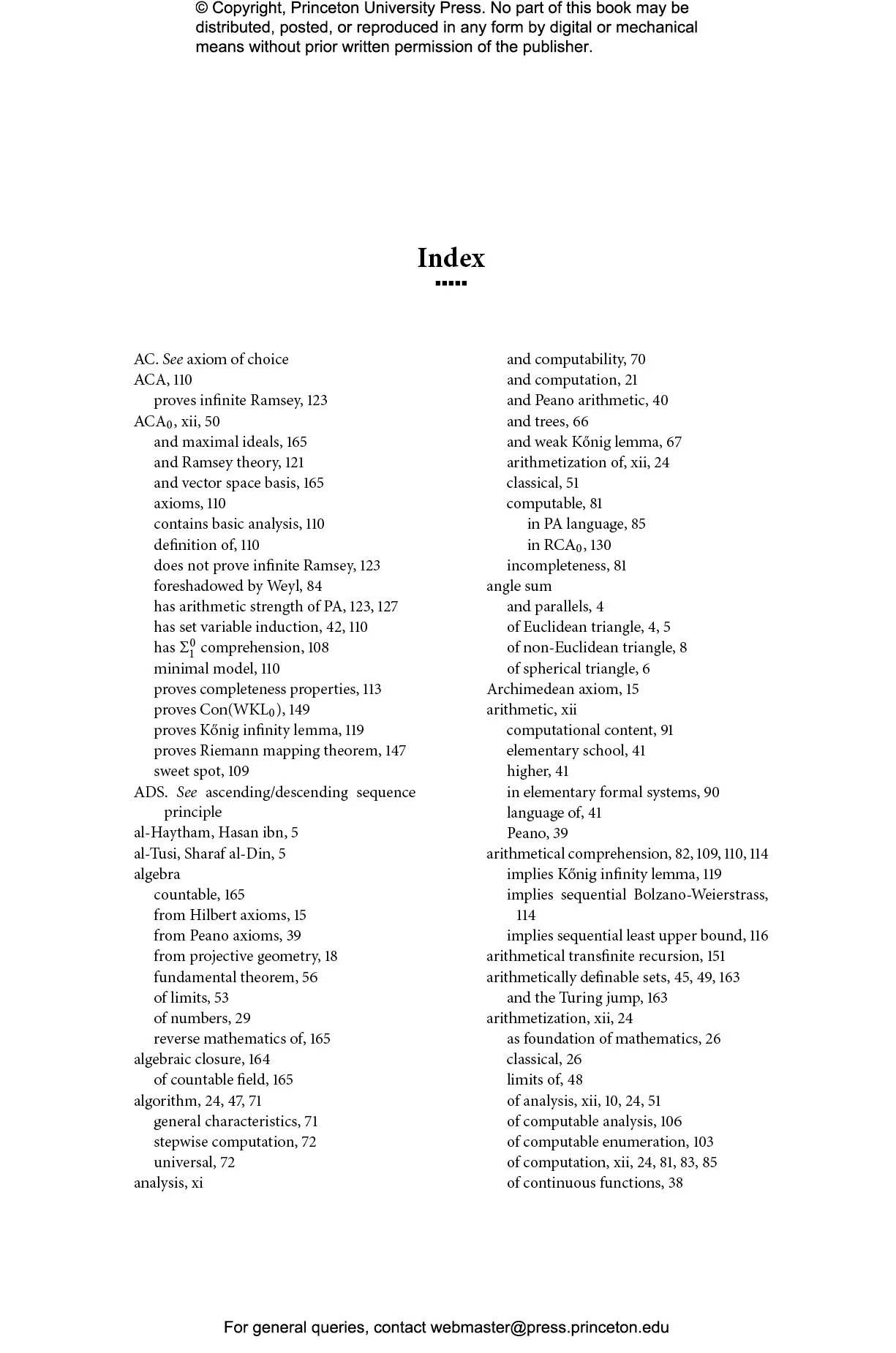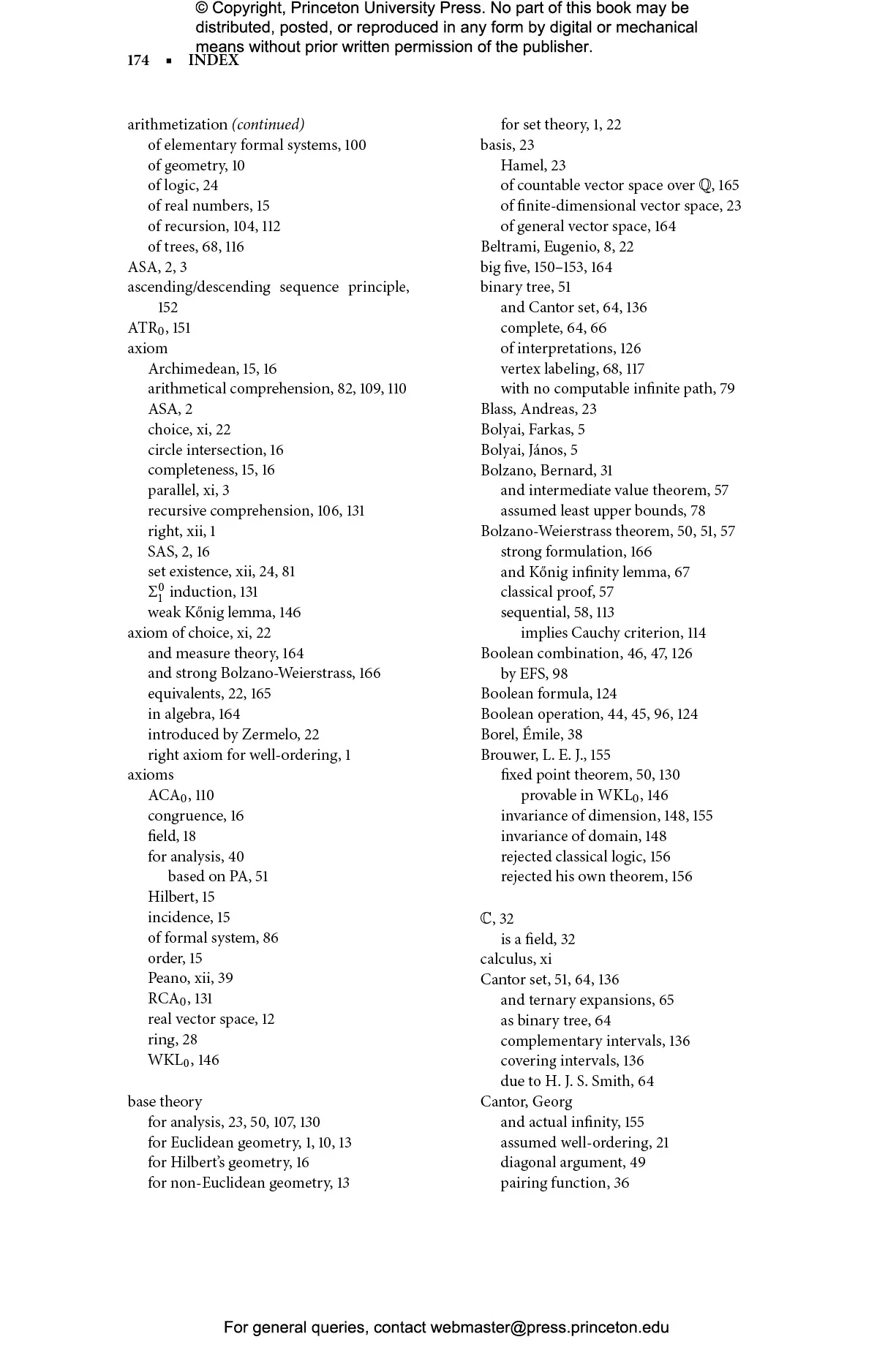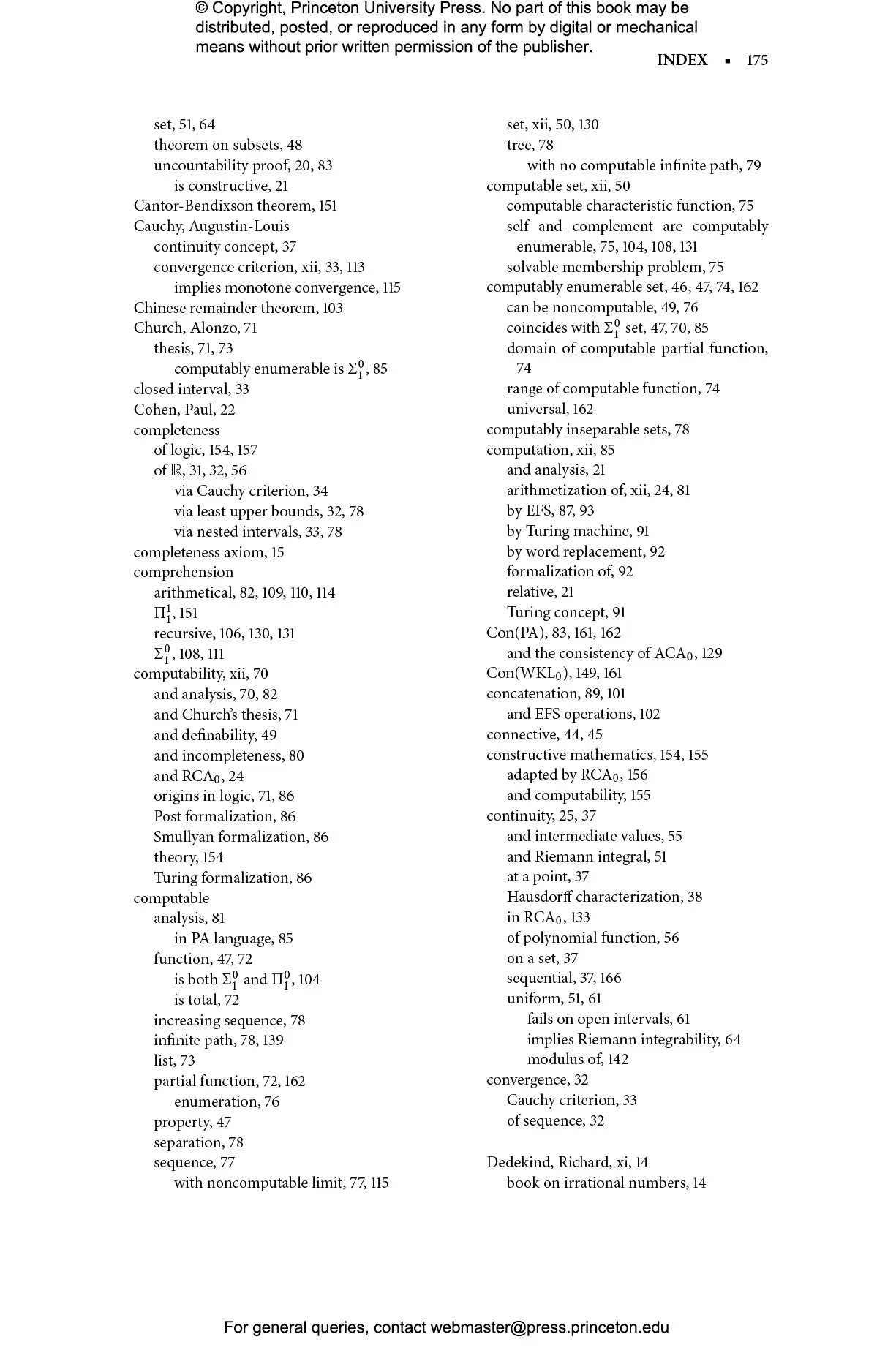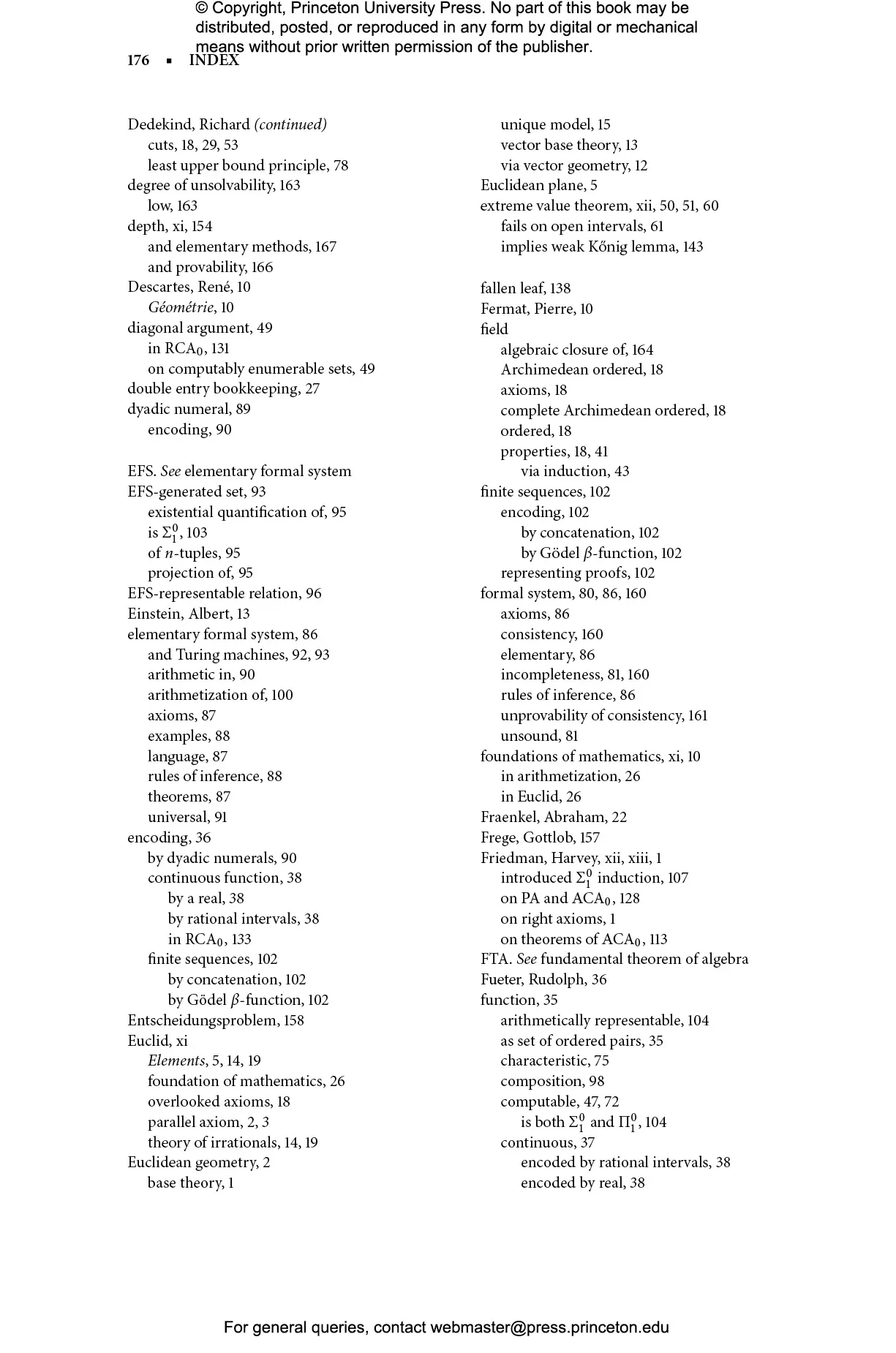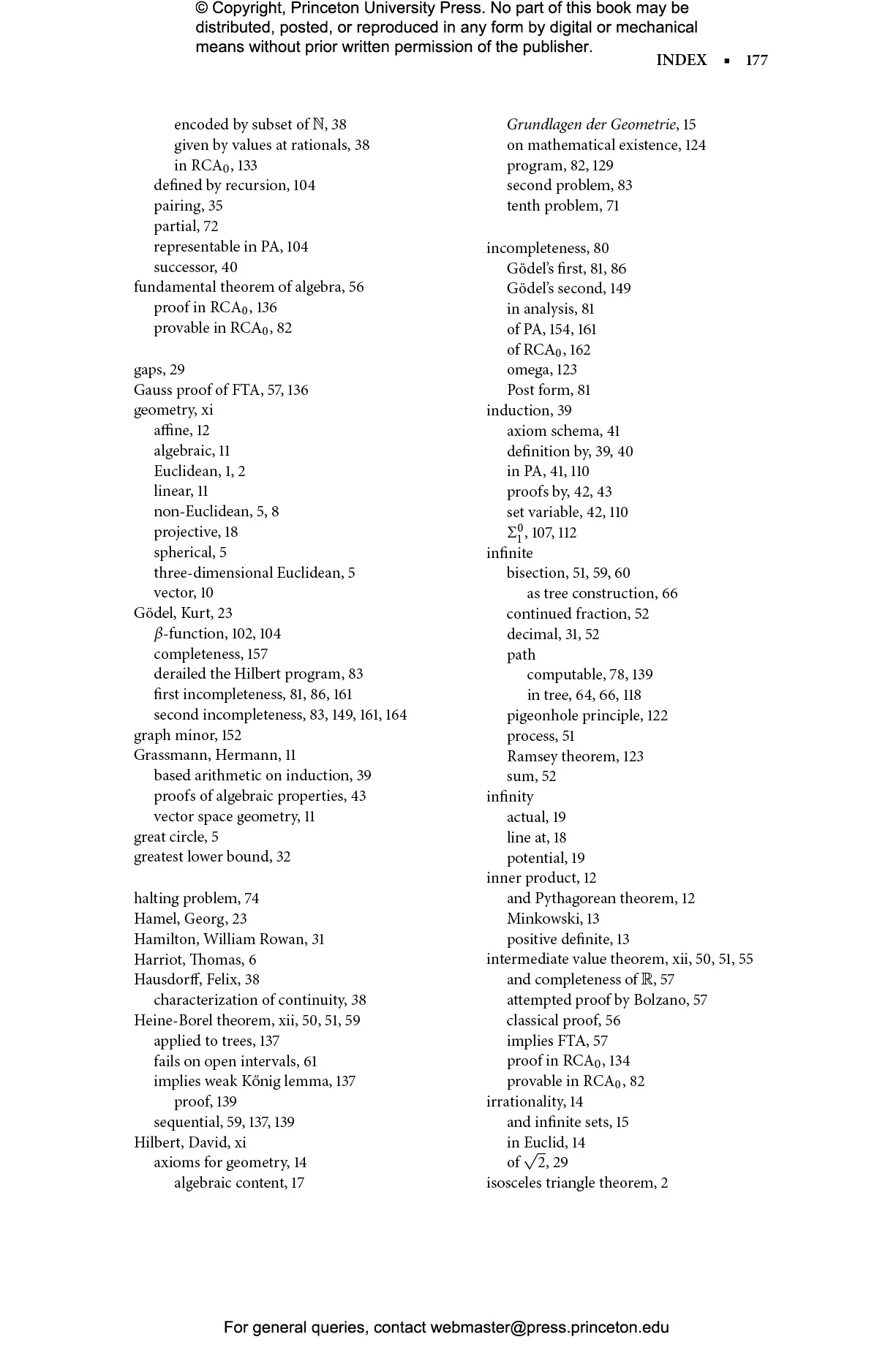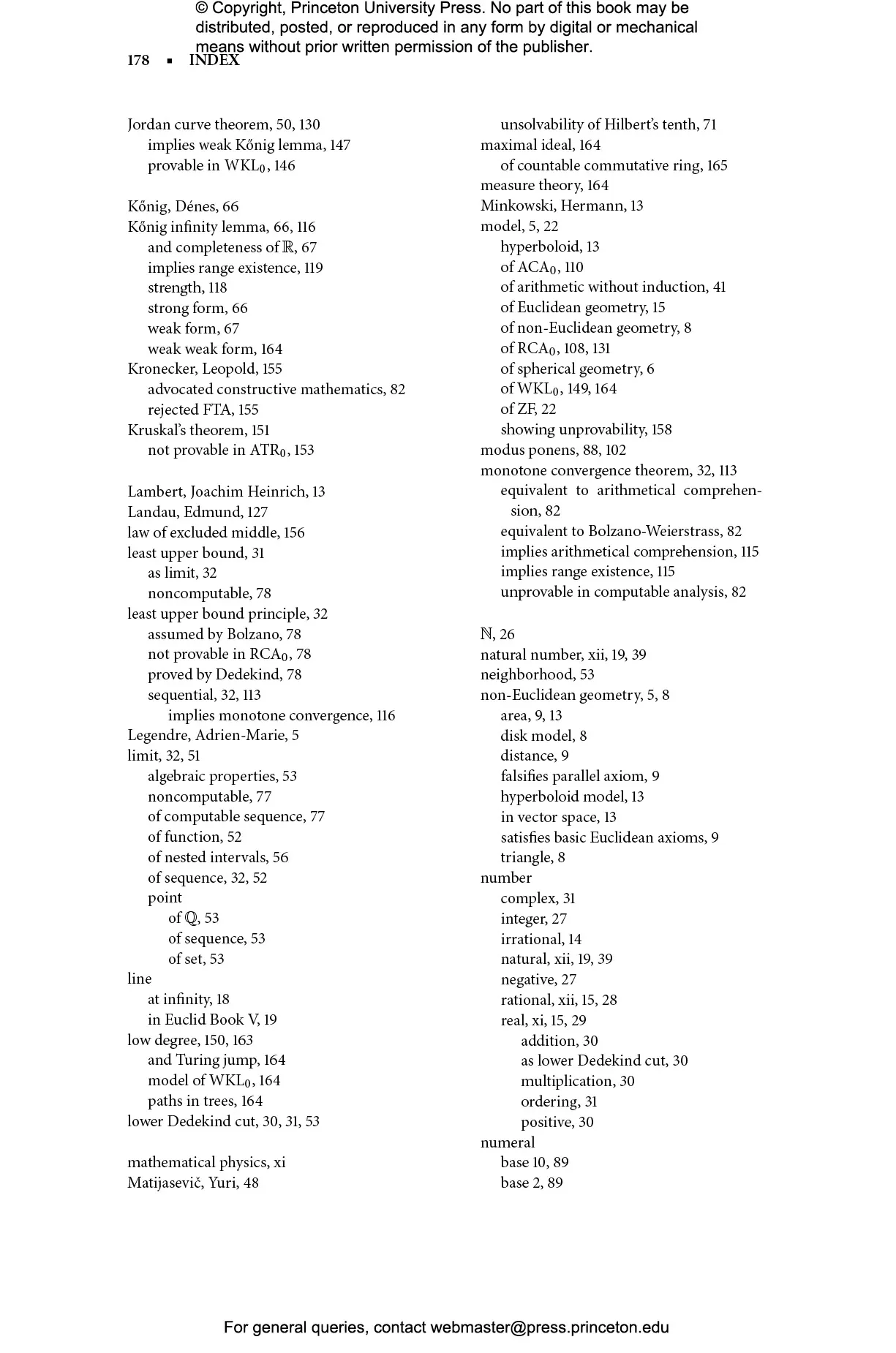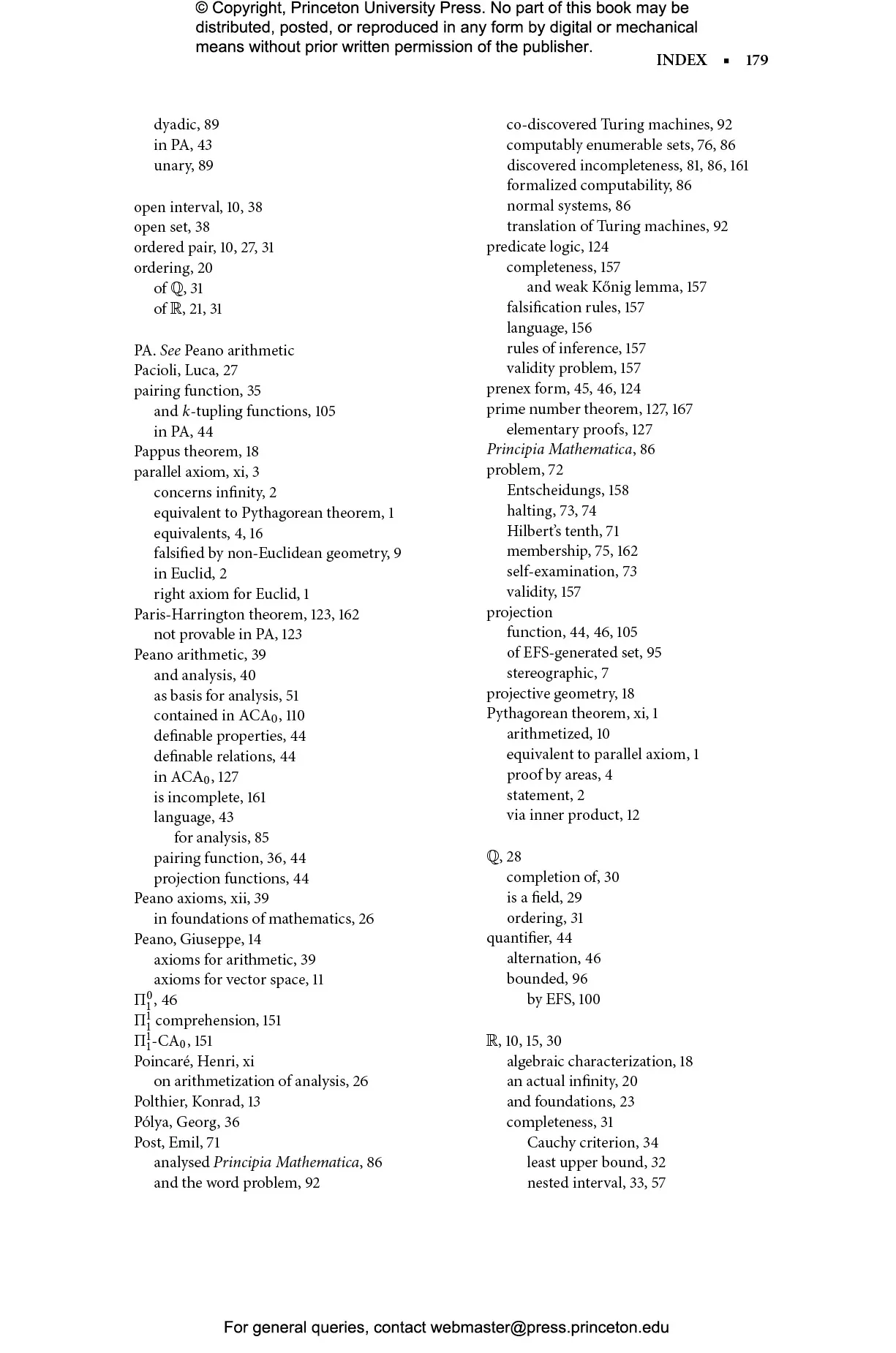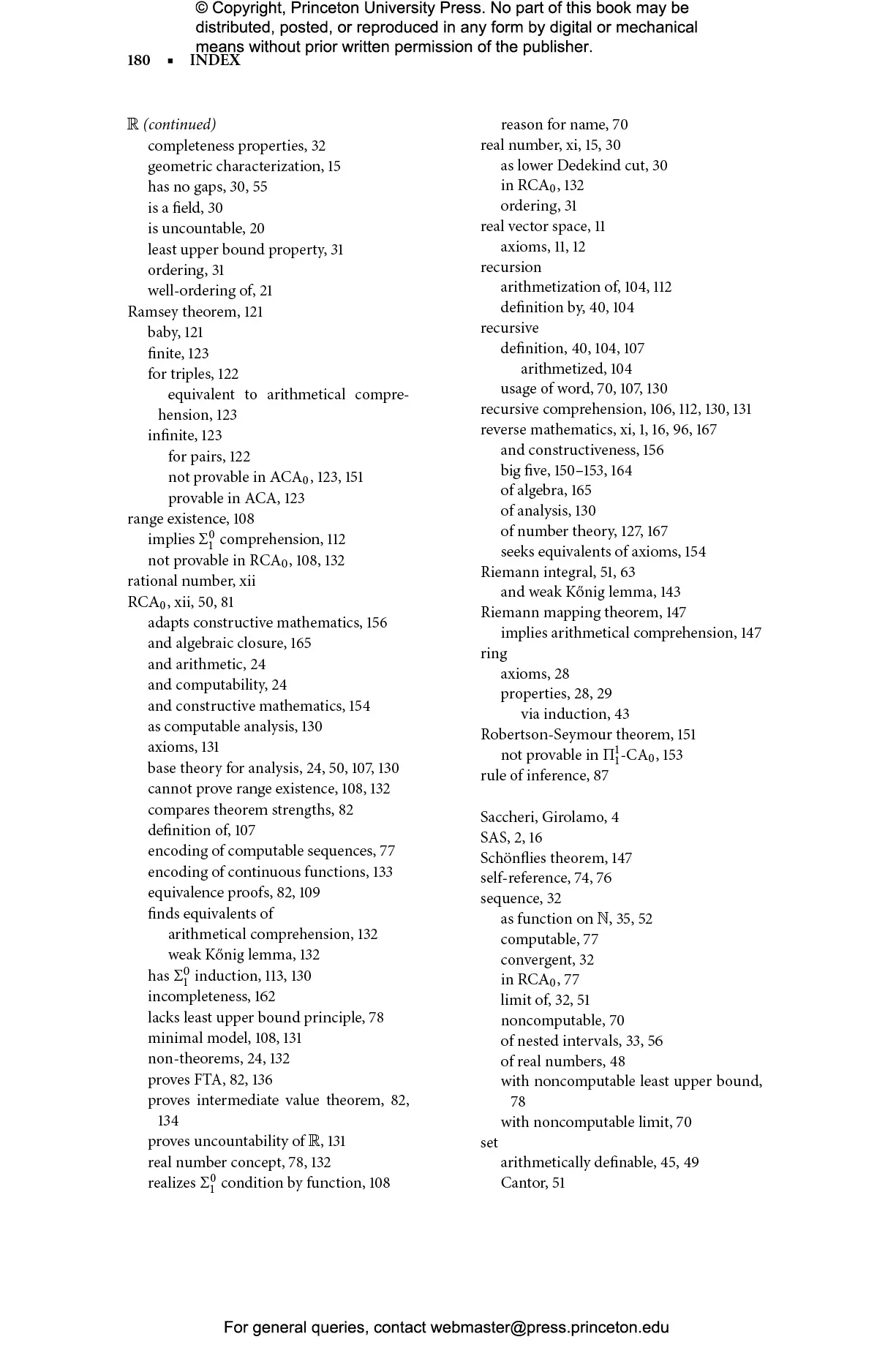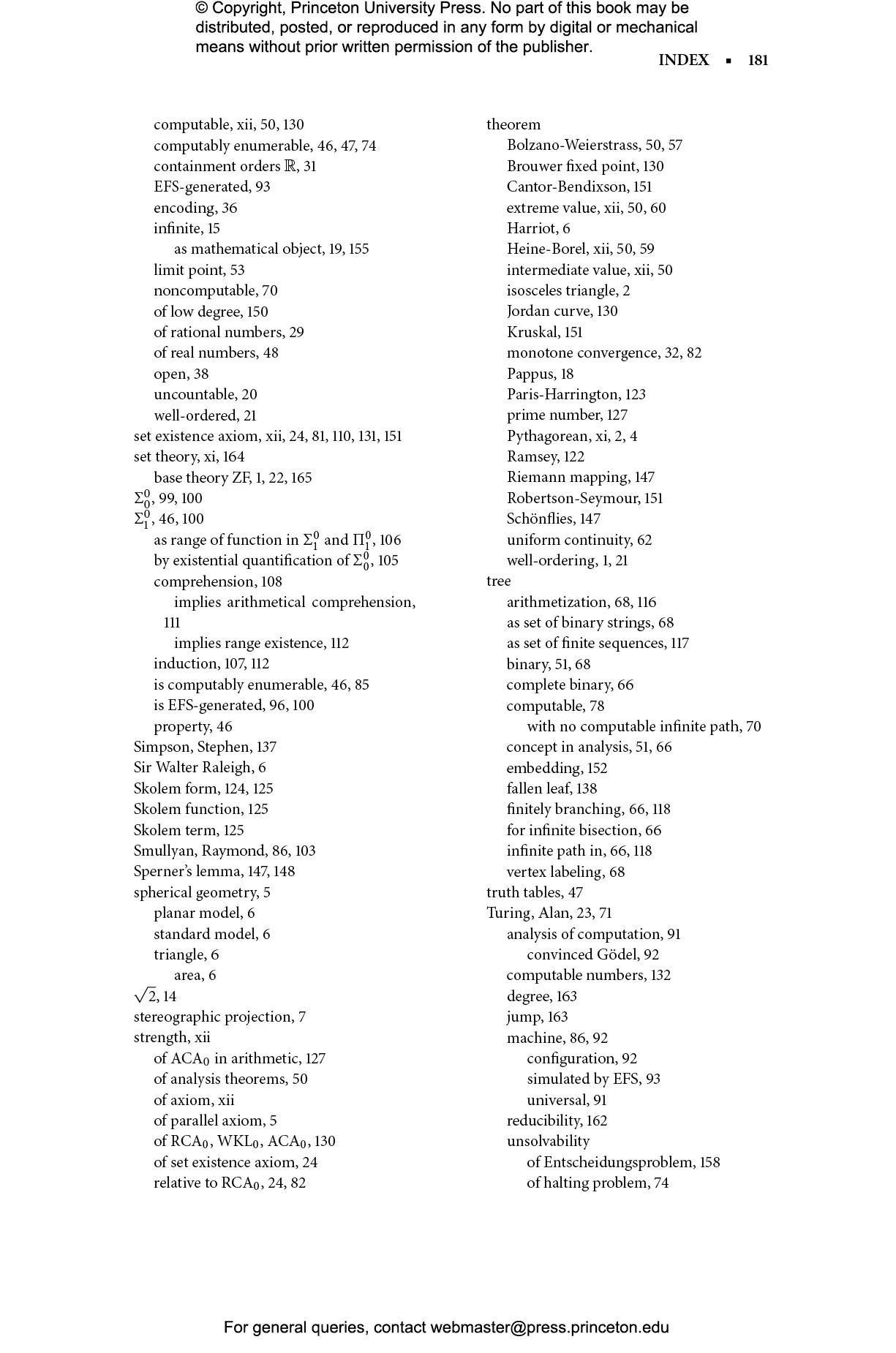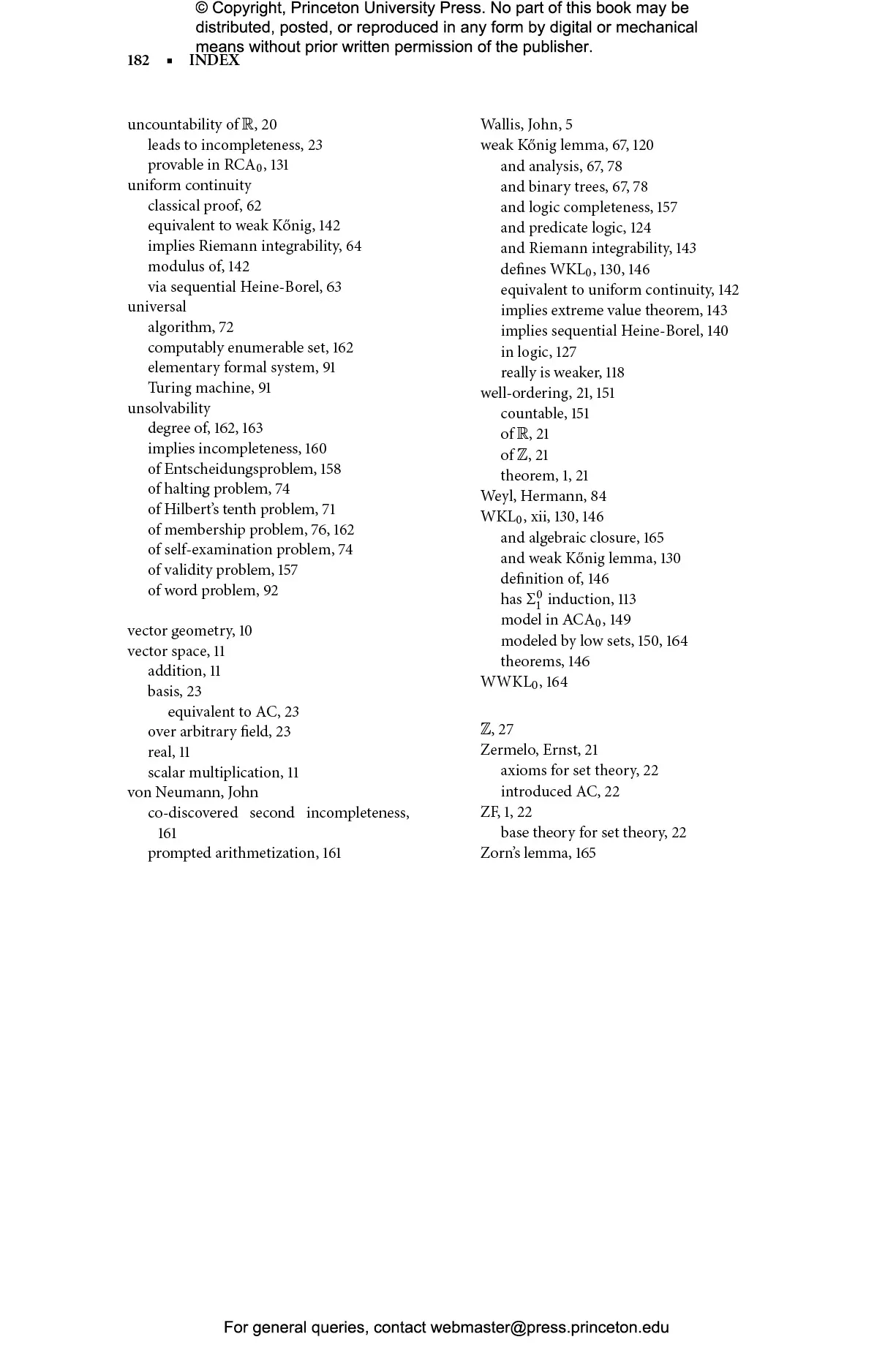Reverse Mathematics

John Stillwell

ebooks

Many of our ebooks are available for purchase from these online vendors:

Many of our ebooks are available through library electronic resources including these platforms:

Reverse Mathematics: Proofs from the Inside Outebook

ISBN:
Published:
Jan 1, 2018
2018
Illus:
5 halftones. 30 line illus.

This book presents reverse mathematics to a general mathematical audience for the first time. Reverse mathematics is a new field that answers some old questions. In the two thousand years that mathematicians have been deriving theorems from axioms, it has often been asked: which axioms are needed to prove a given theorem? Only in the last two hundred years have some of these questions been answered, and only in the last forty years has a systematic approach been developed. In Reverse Mathematics, John Stillwell gives a representative view of this field, emphasizing basic analysis—finding the “right axioms” to prove fundamental theorems—and giving a novel approach to logic.

Stillwell introduces reverse mathematics historically, describing the two developments that made reverse mathematics possible, both involving the idea of arithmetization. The first was the nineteenth-century project of arithmetizing analysis, which aimed to define all concepts of analysis in terms of natural numbers and sets of natural numbers. The second was the twentieth-century arithmetization of logic and computation. Thus arithmetic in some sense underlies analysis, logic, and computation. Reverse mathematics exploits this insight by viewing analysis as arithmetic extended by axioms about the existence of infinite sets. Remarkably, only a small number of axioms are needed for reverse mathematics, and, for each basic theorem of analysis, Stillwell finds the “right axiom” to prove it.

By using a minimum of mathematical logic in a well-motivated way, Reverse Mathematics will engage advanced undergraduates and all mathematicians interested in the foundations of mathematics.